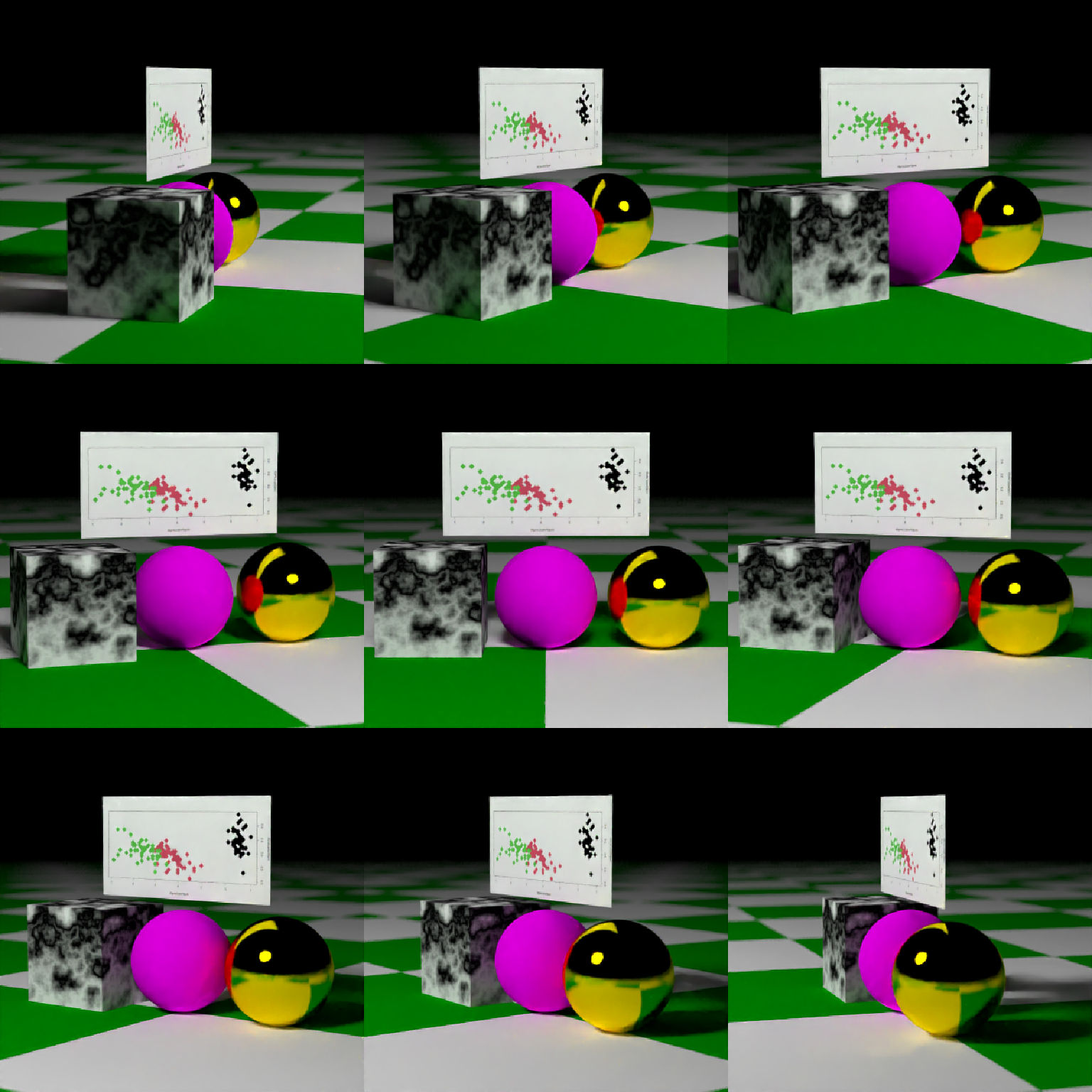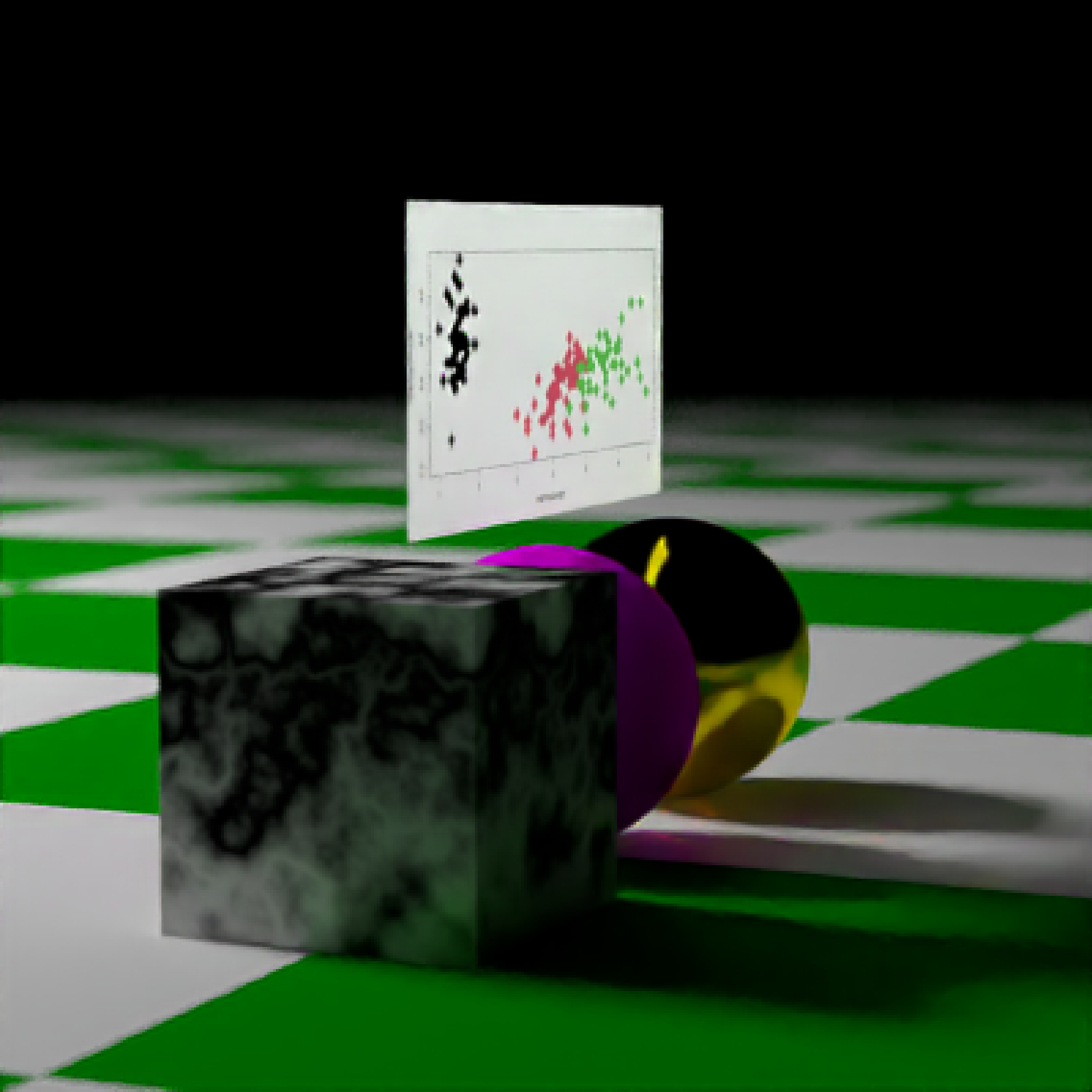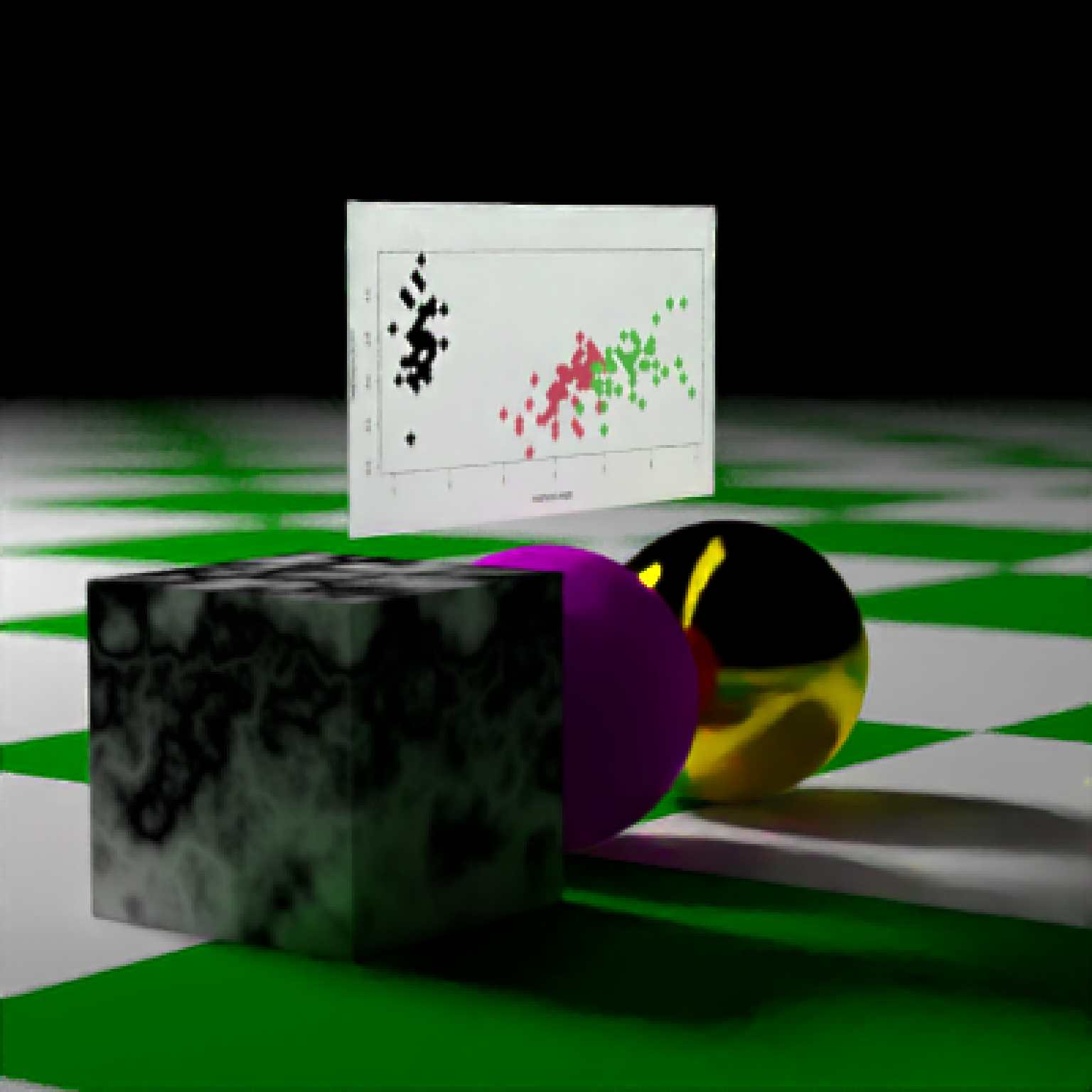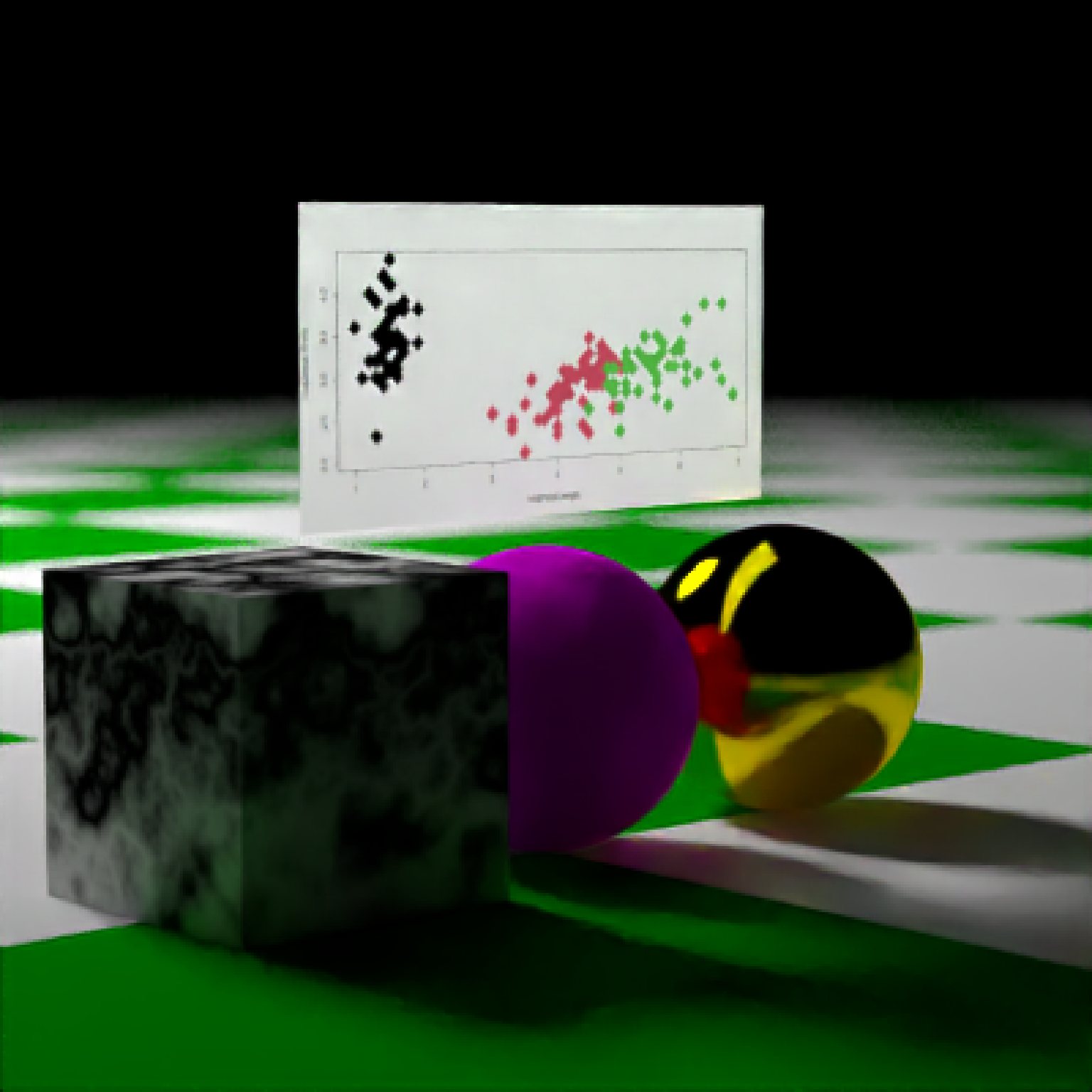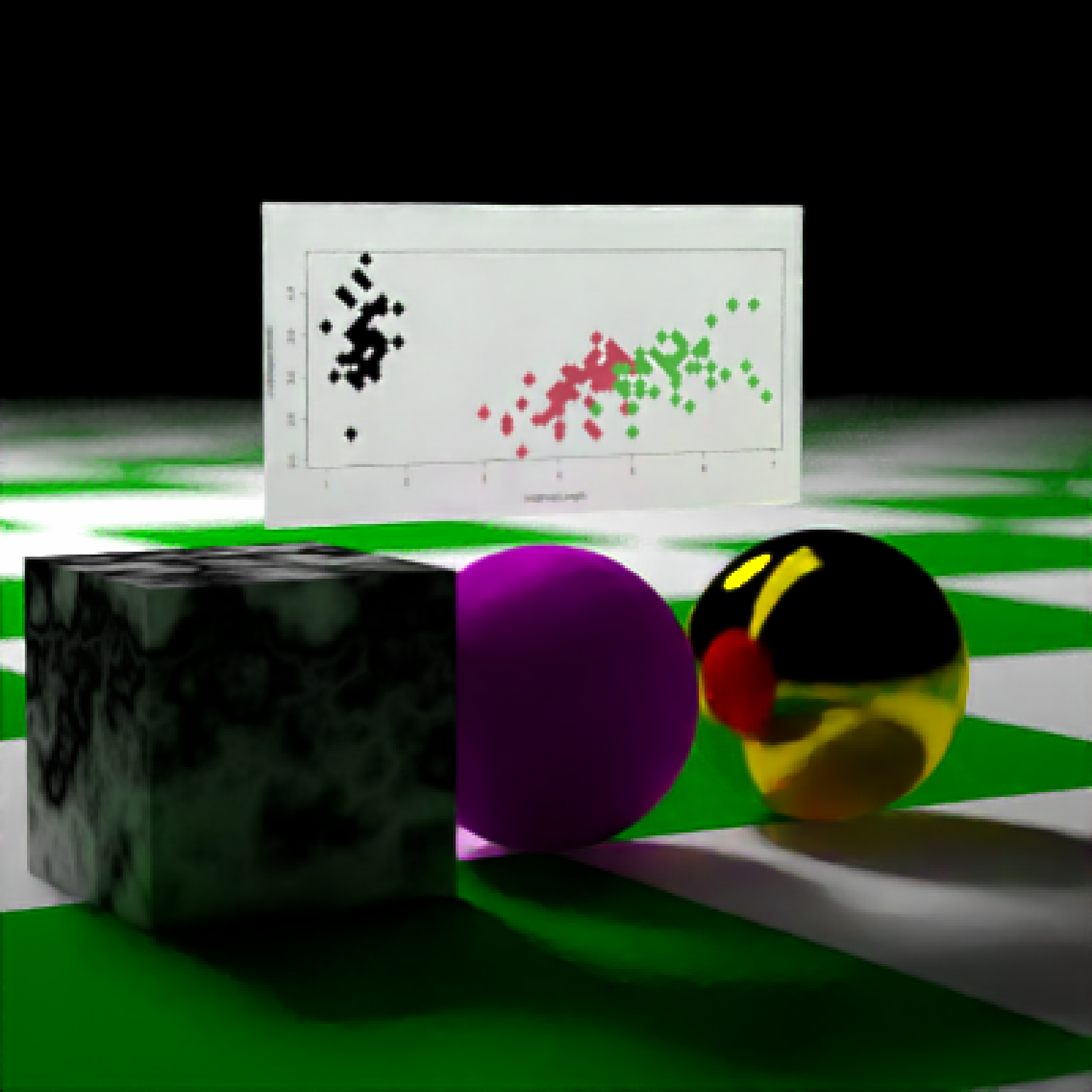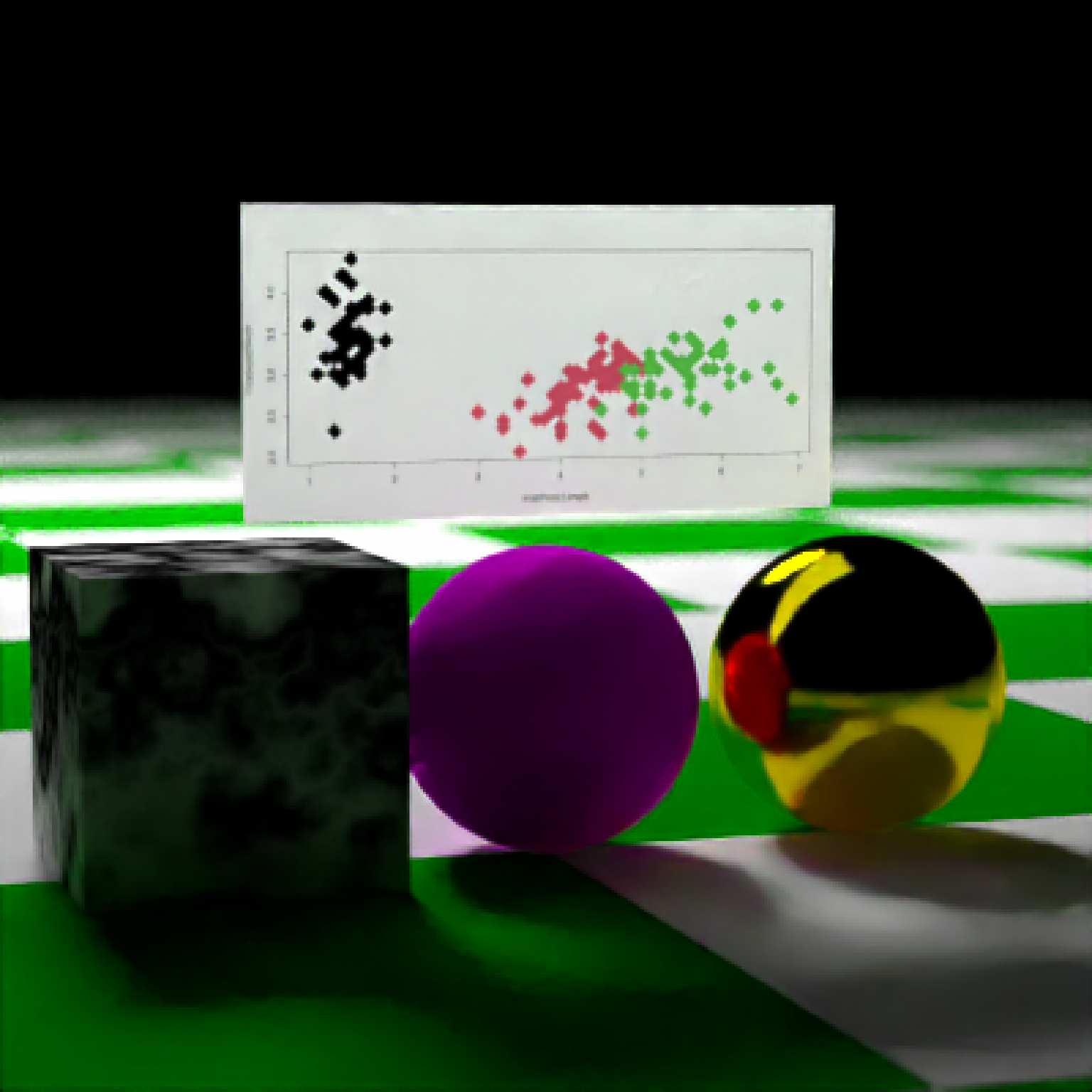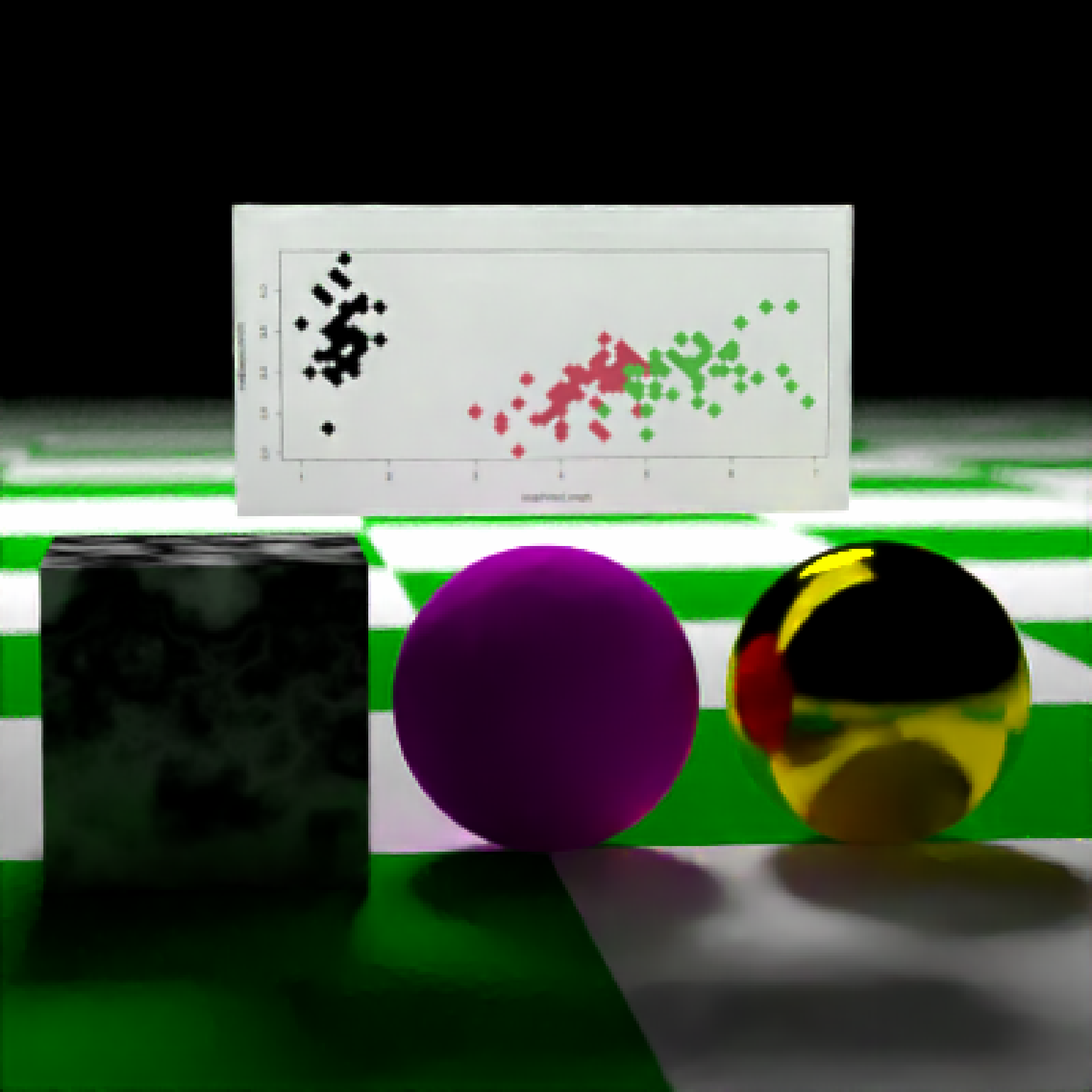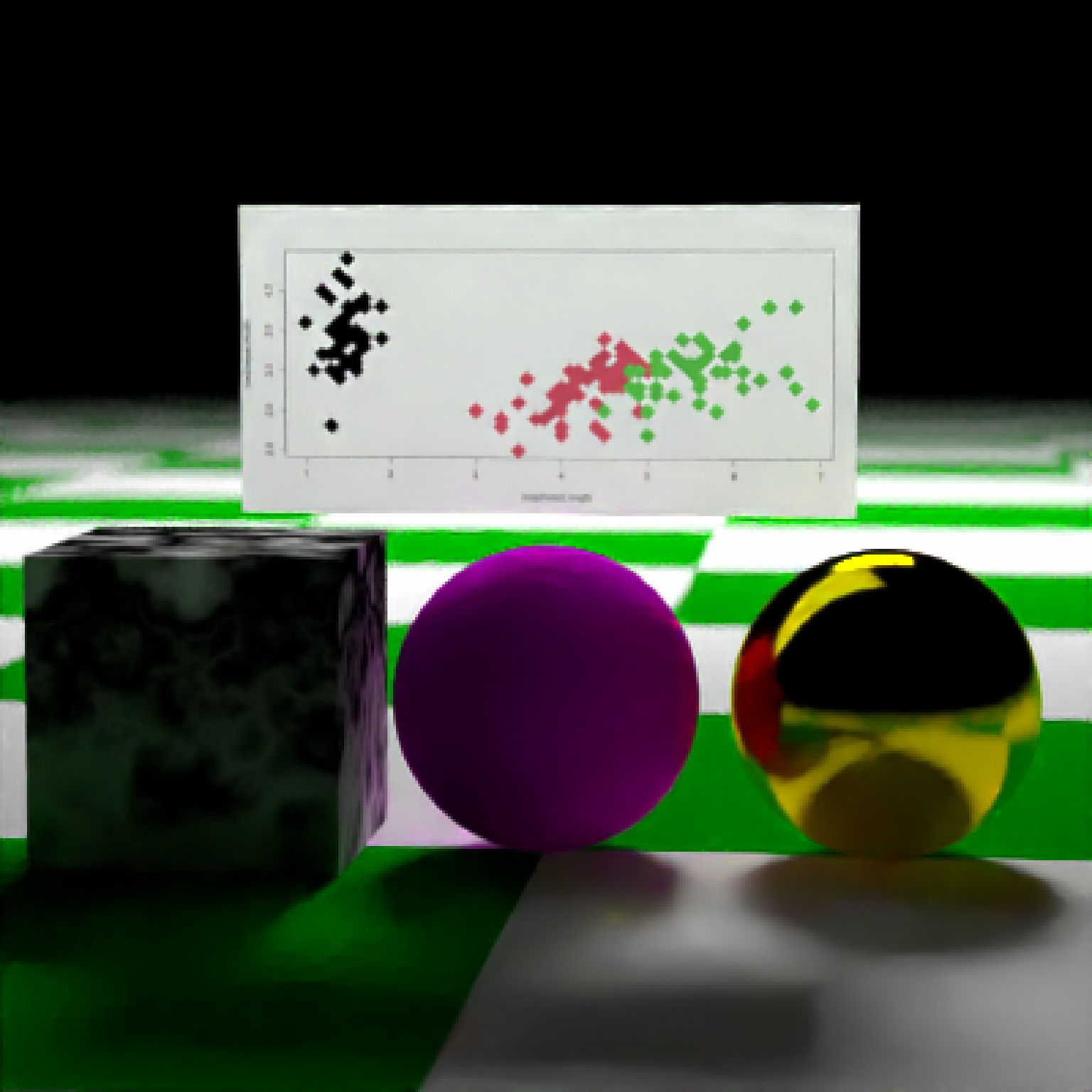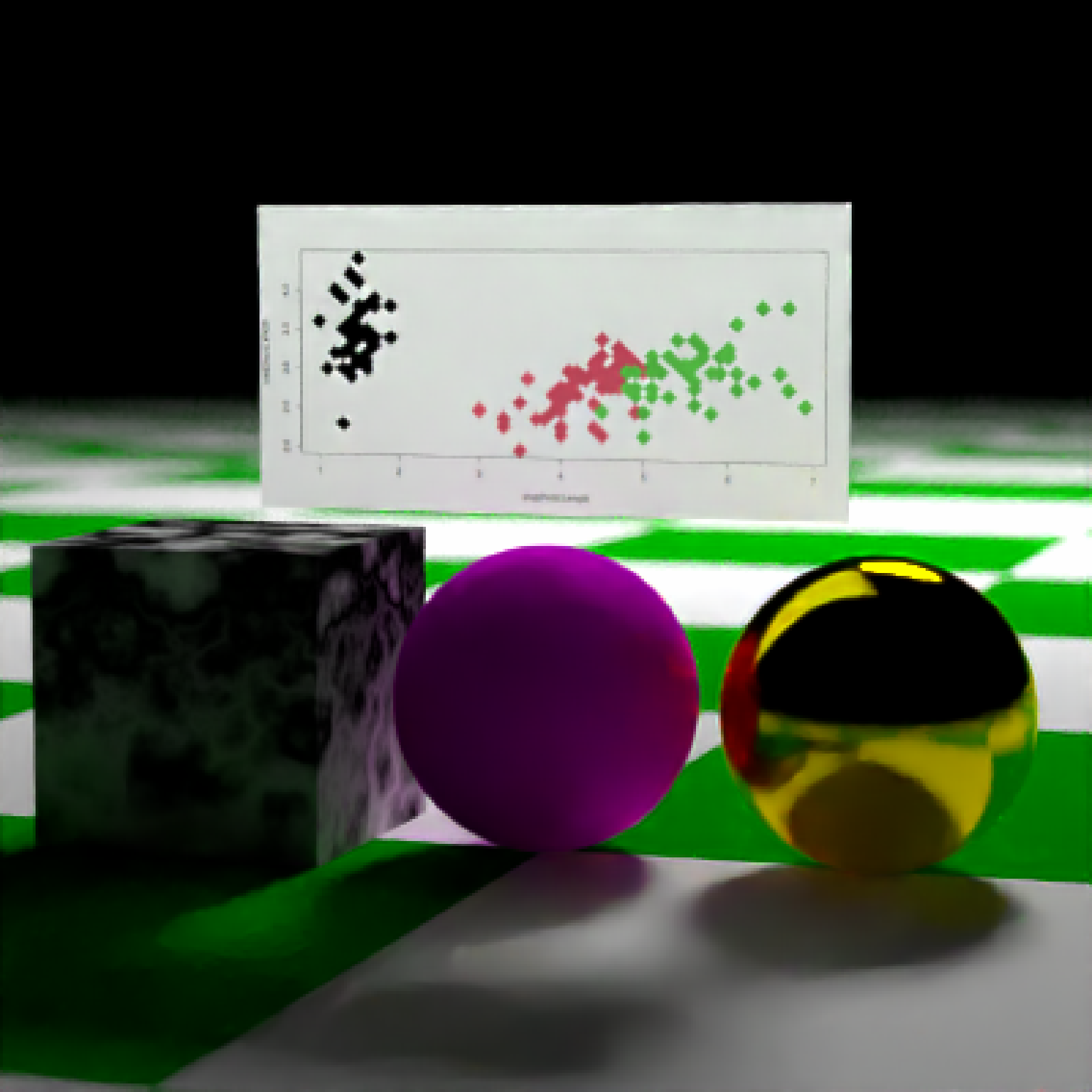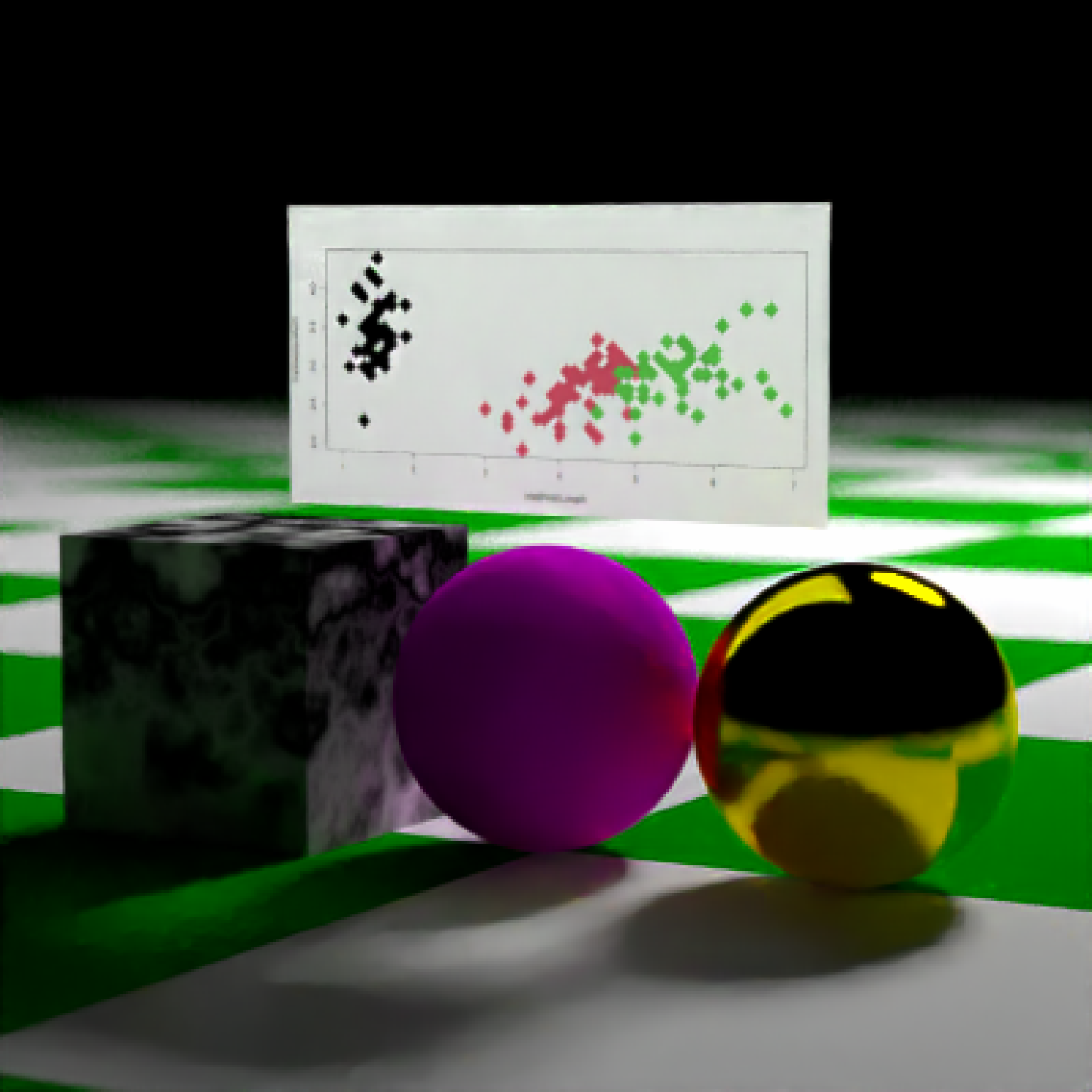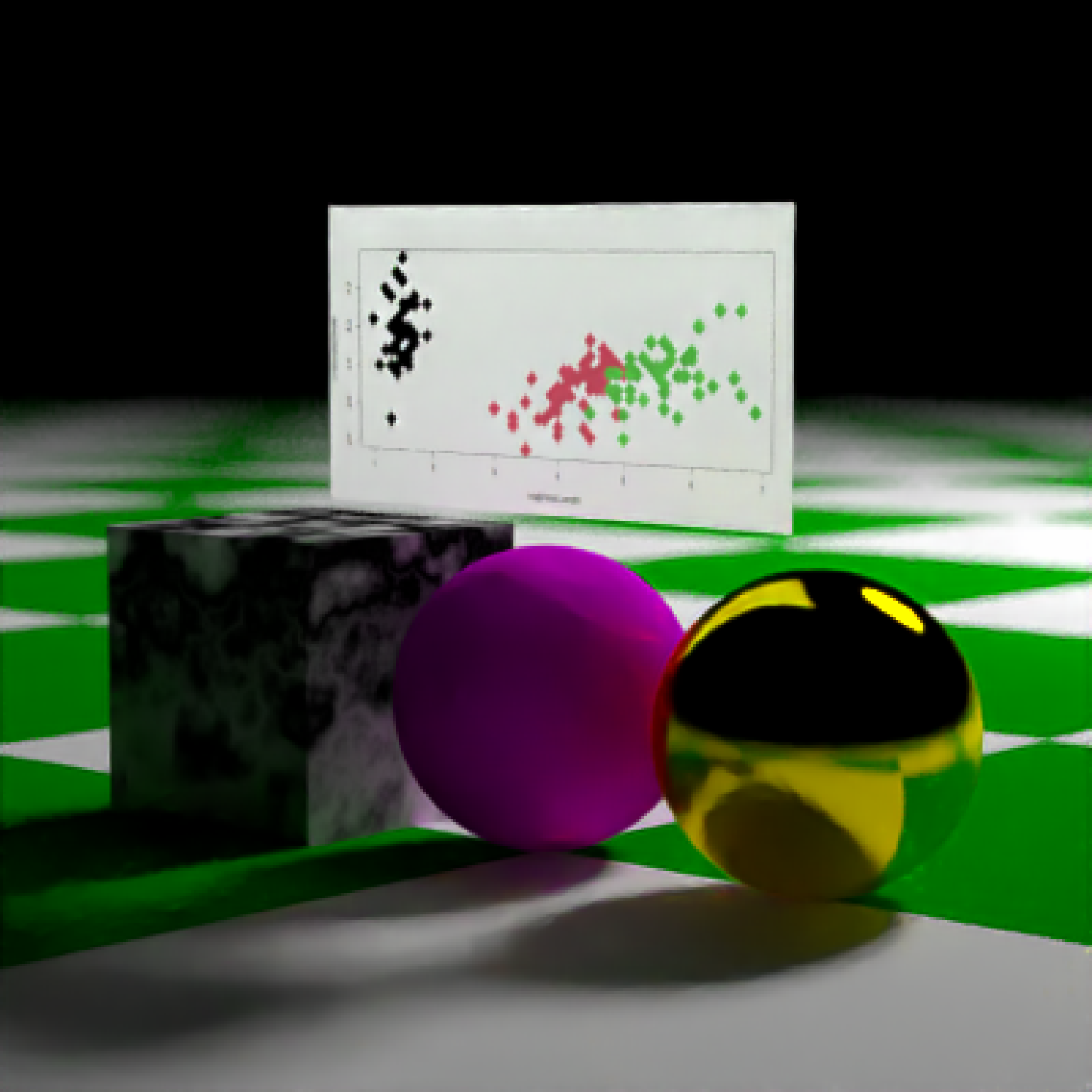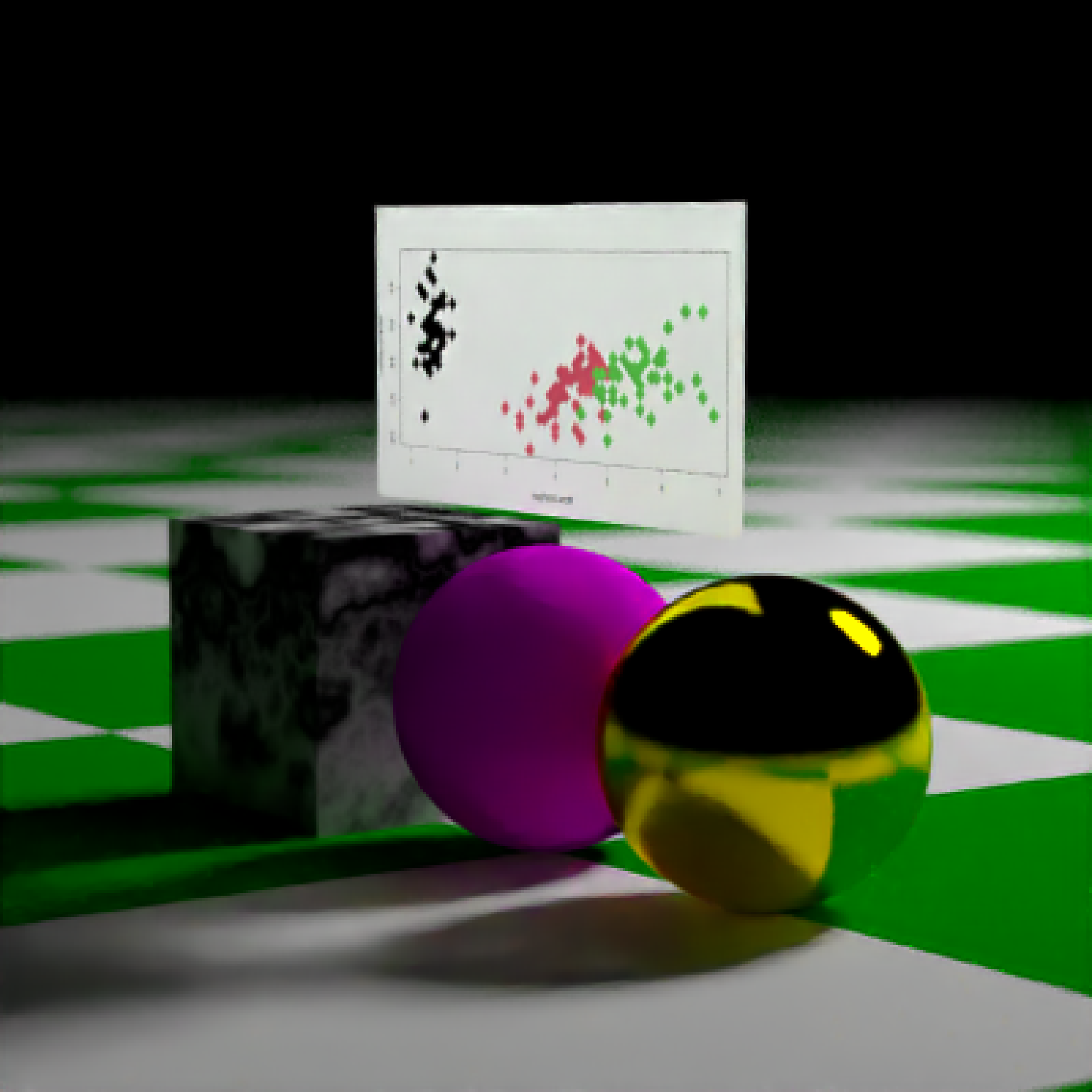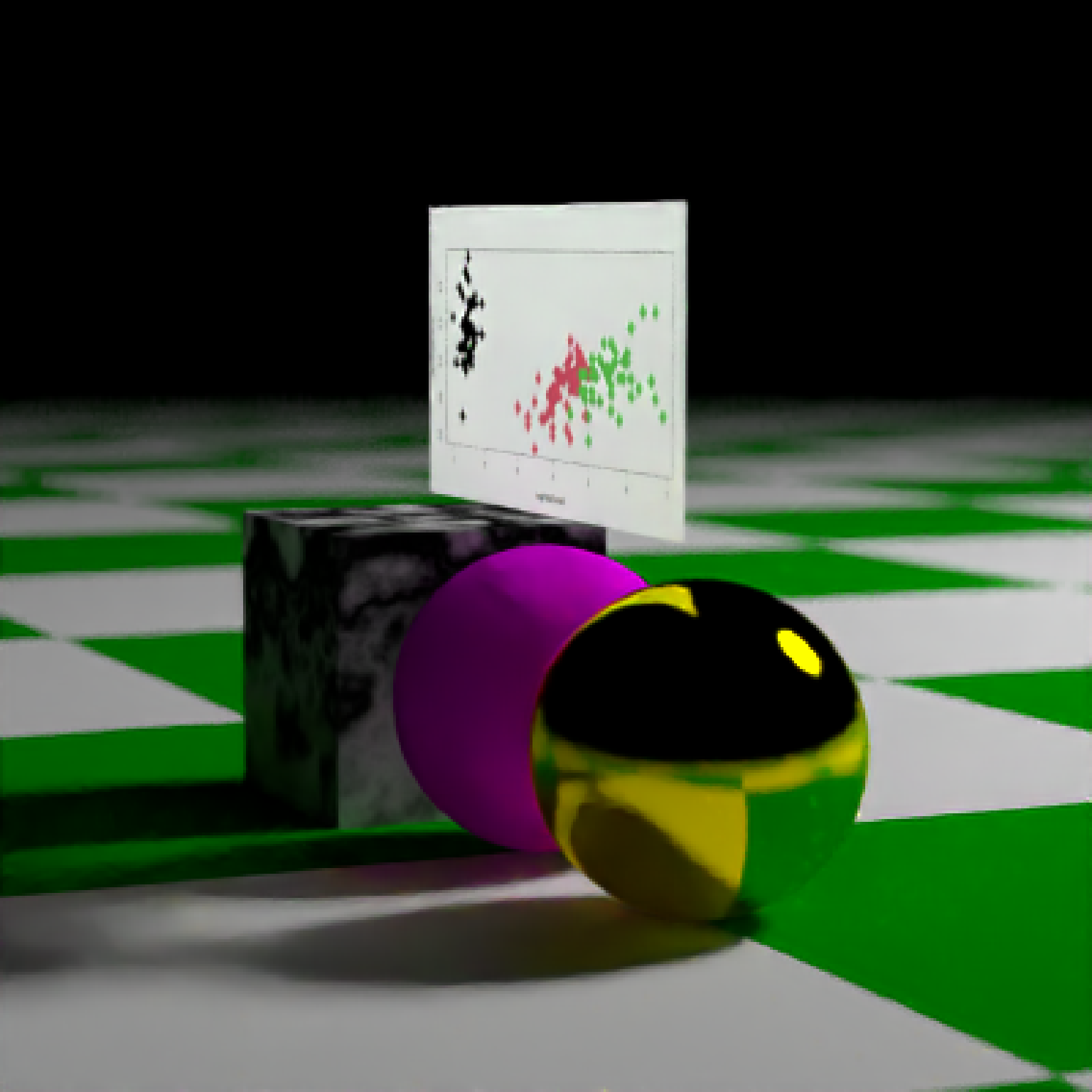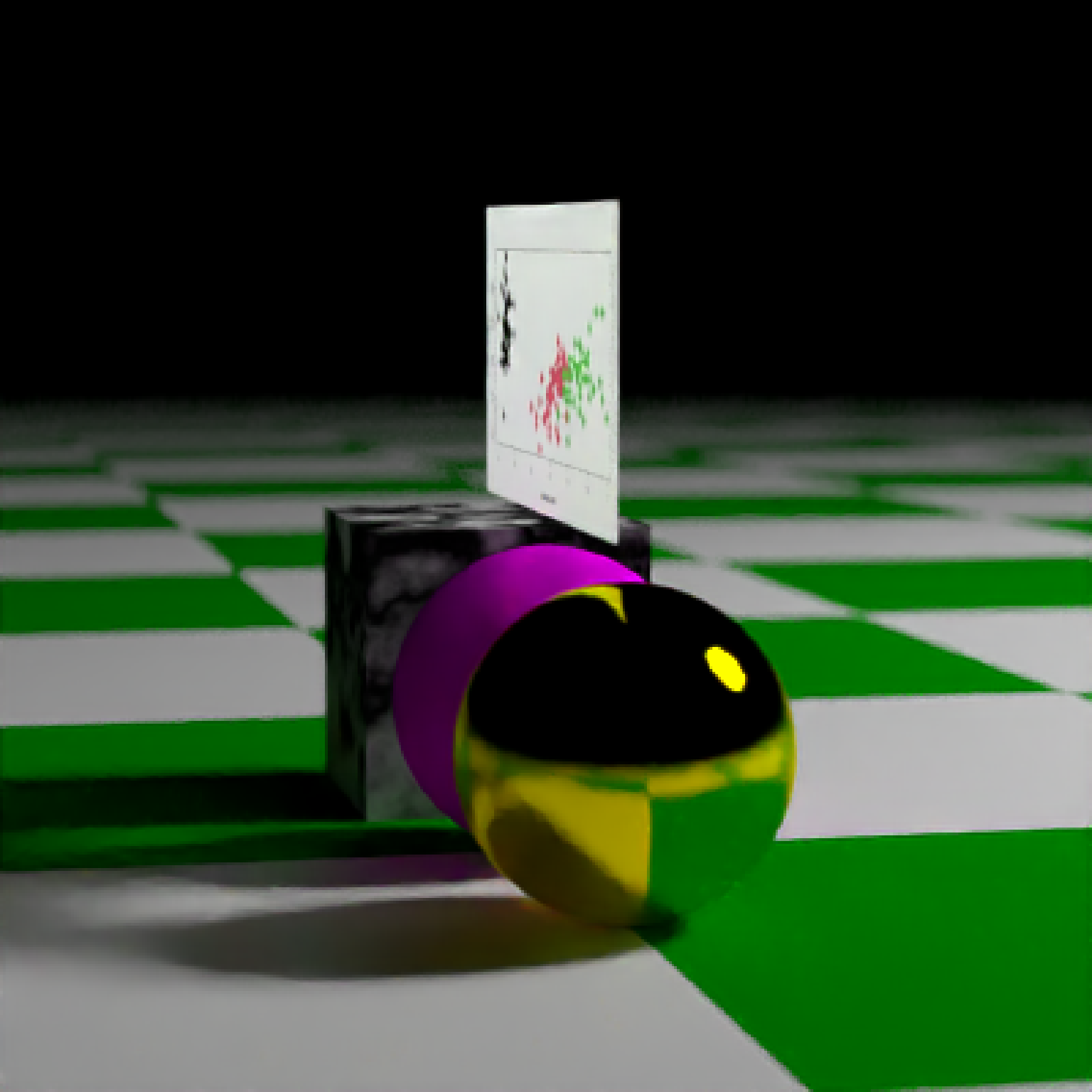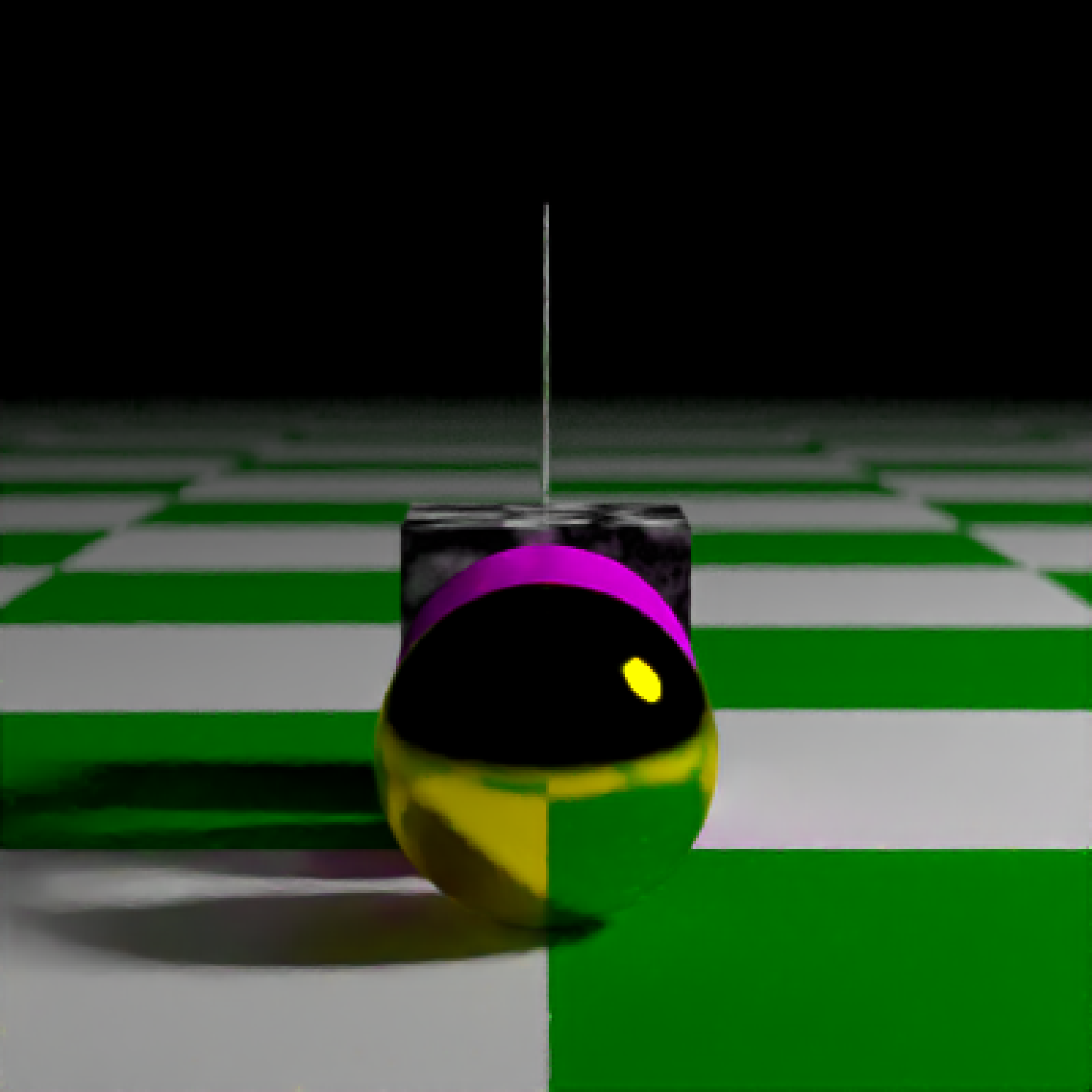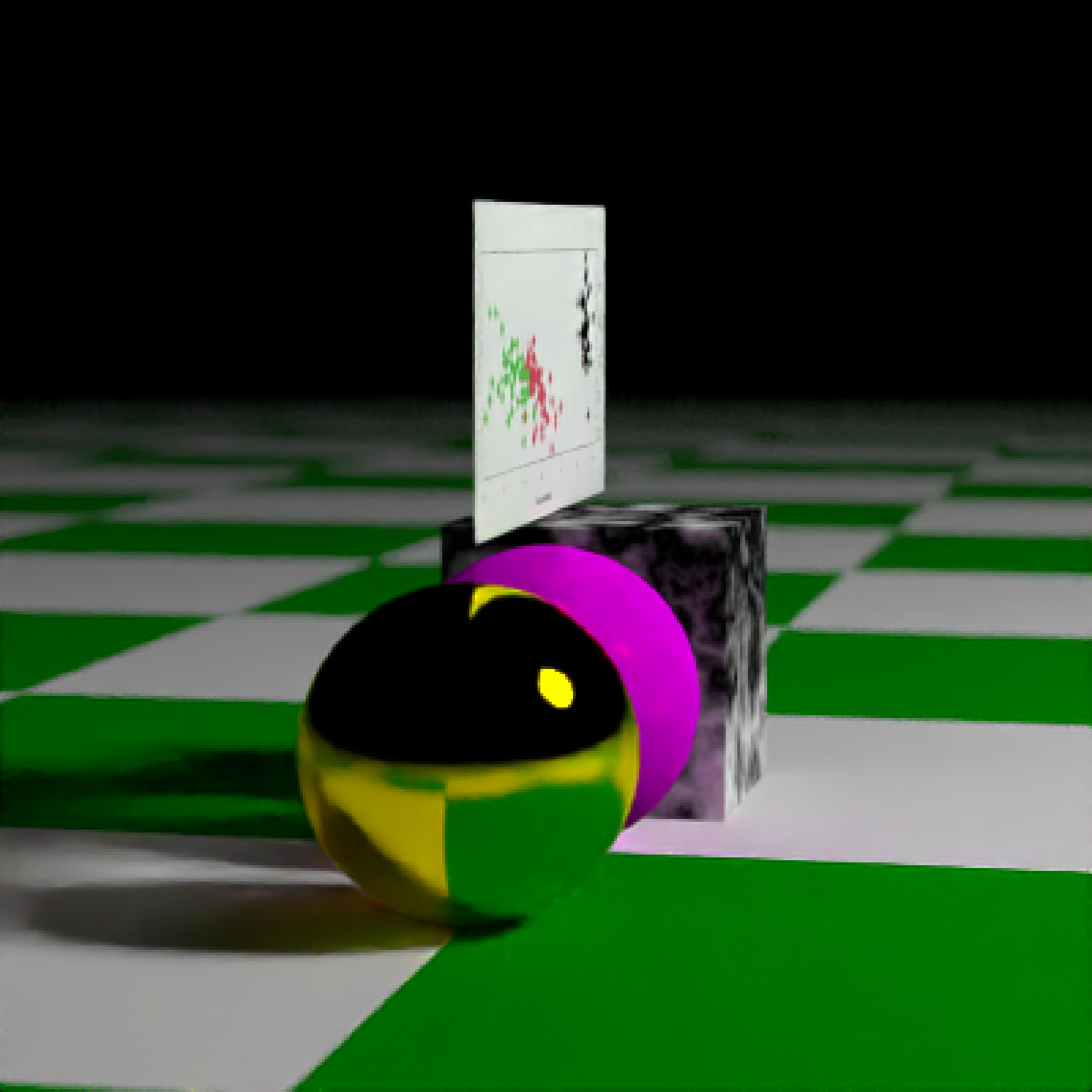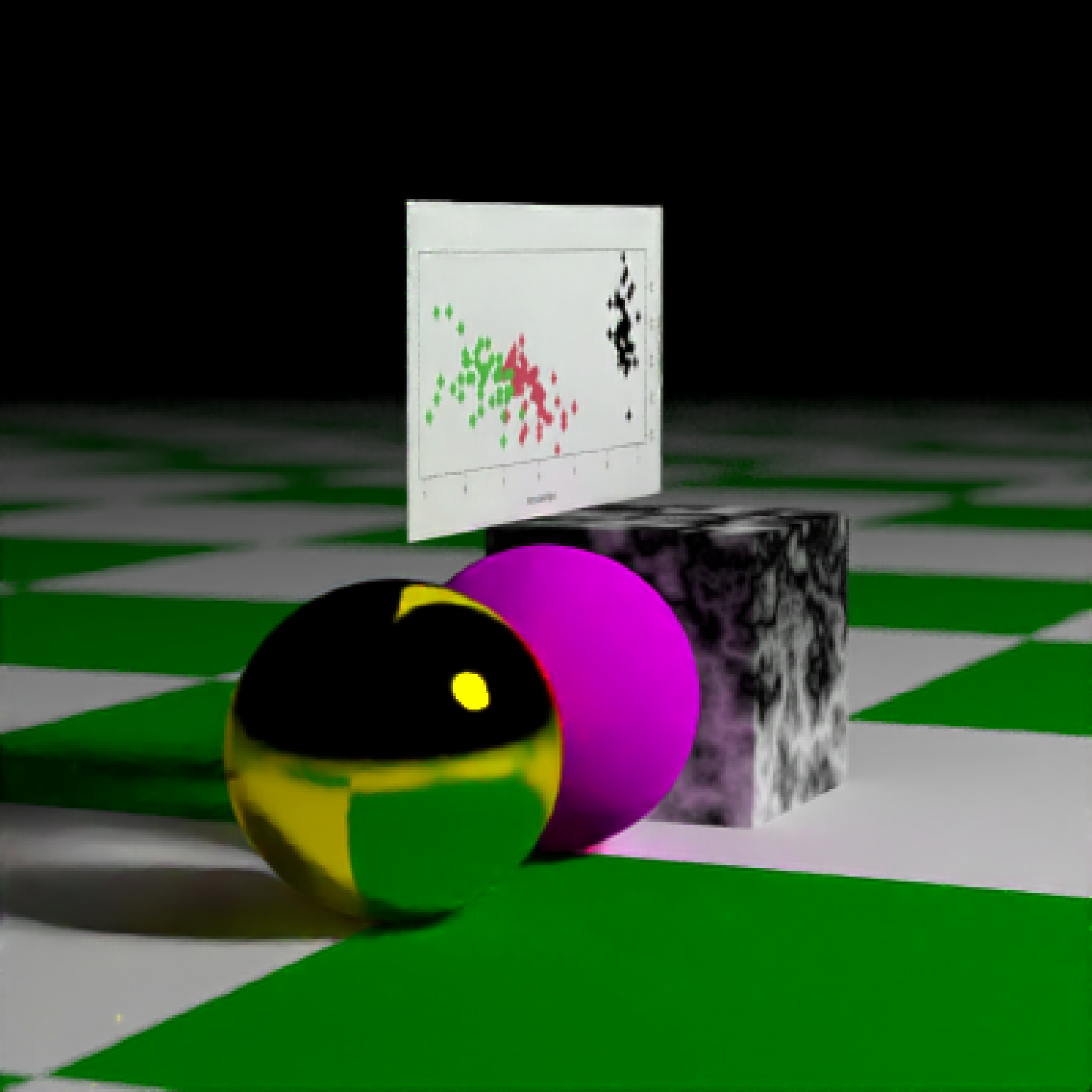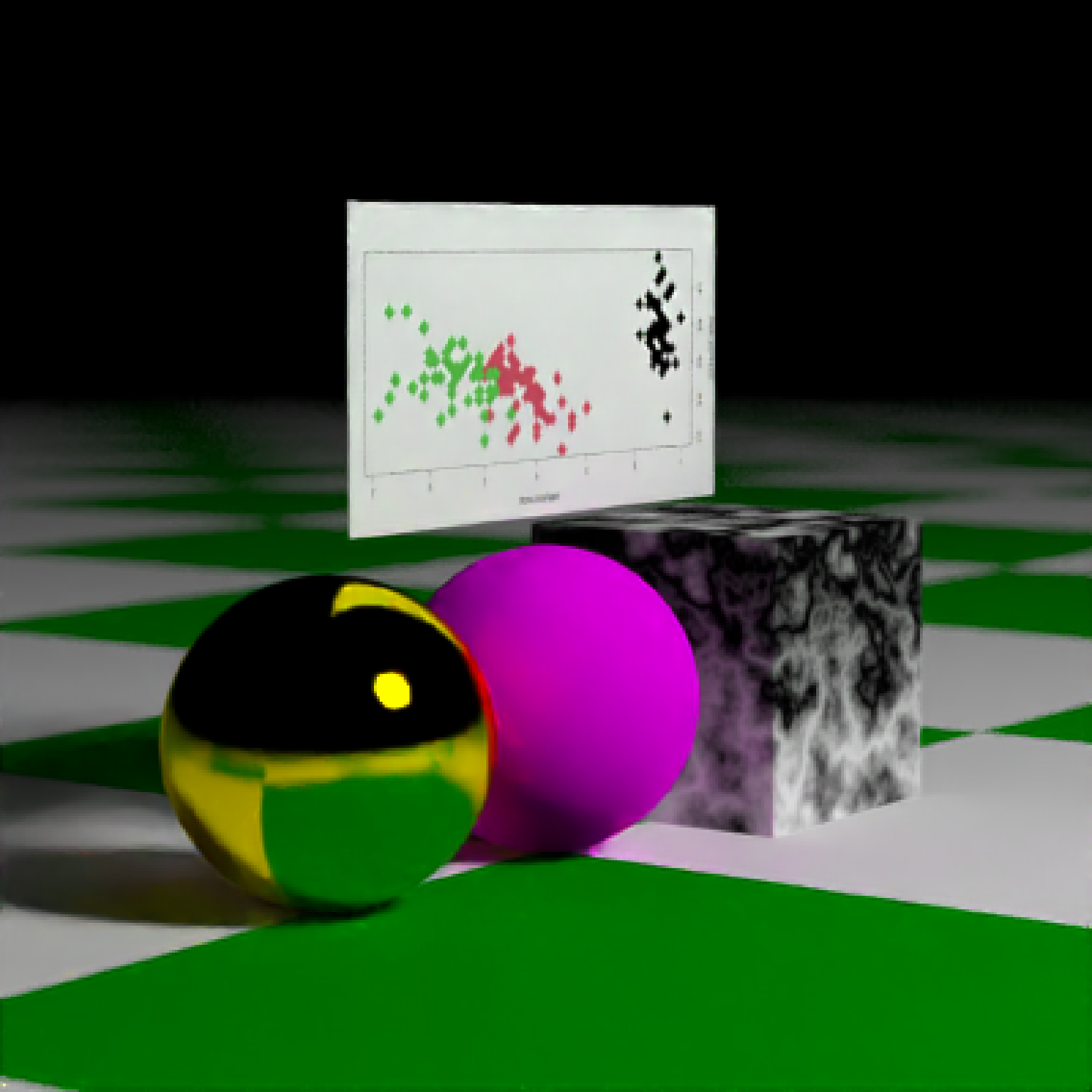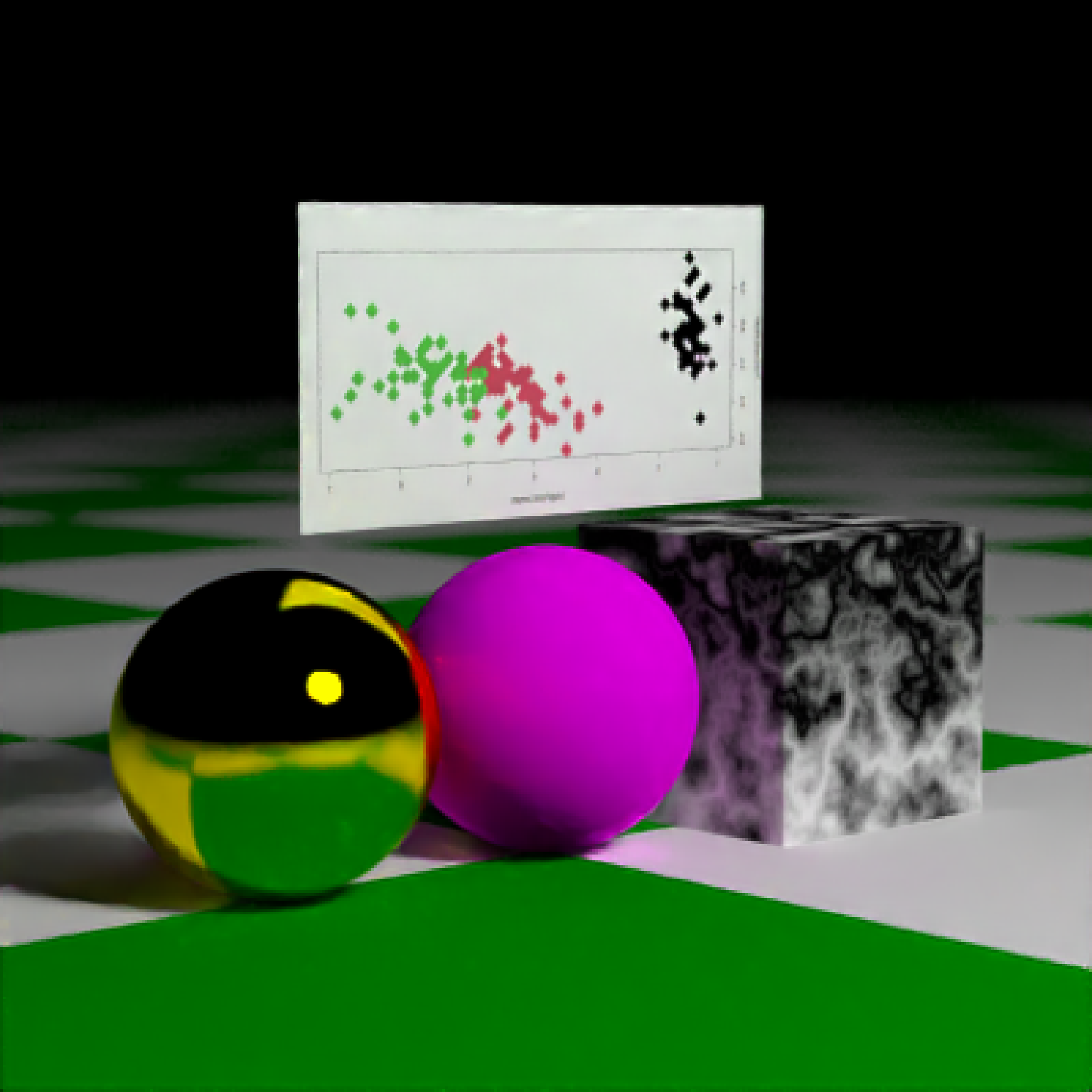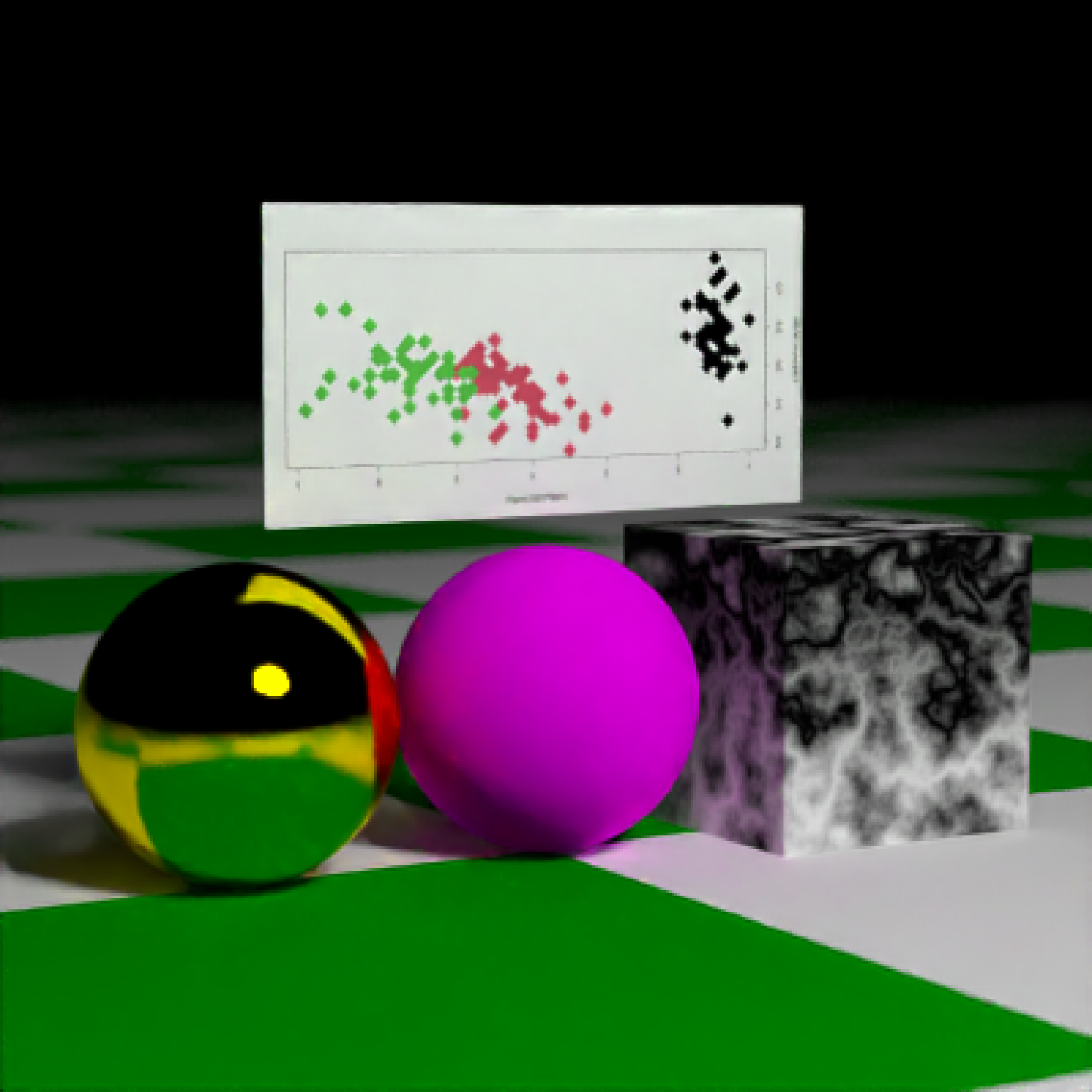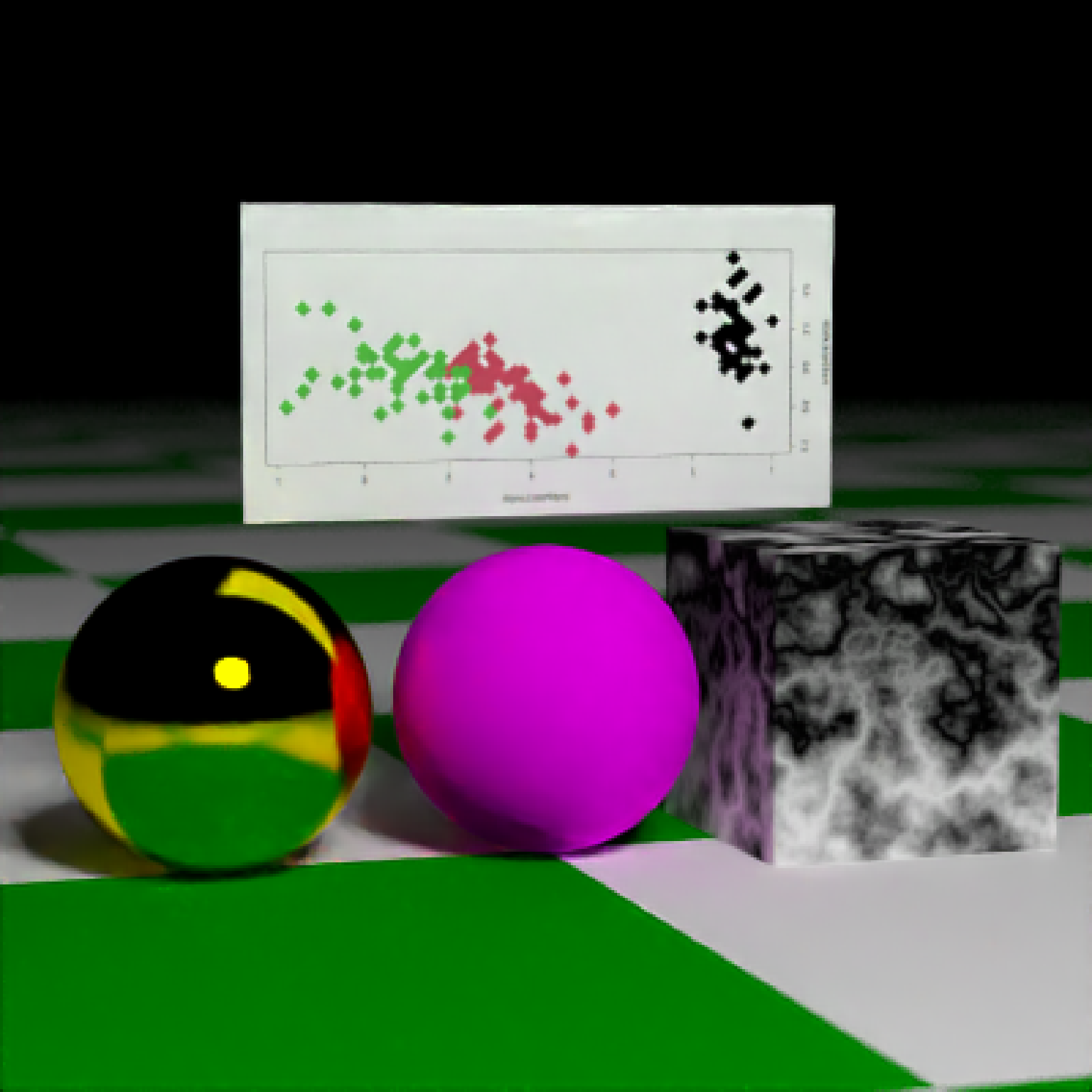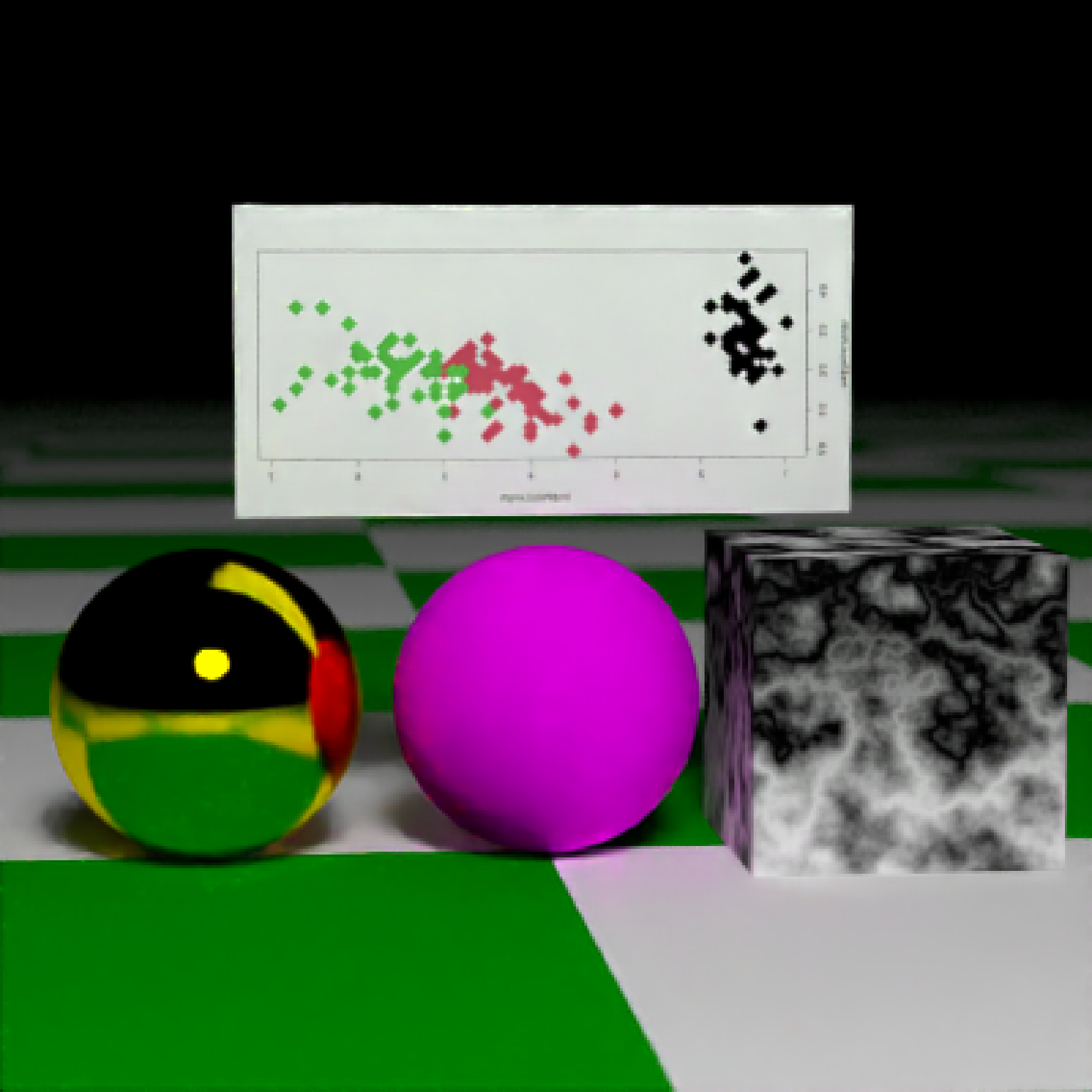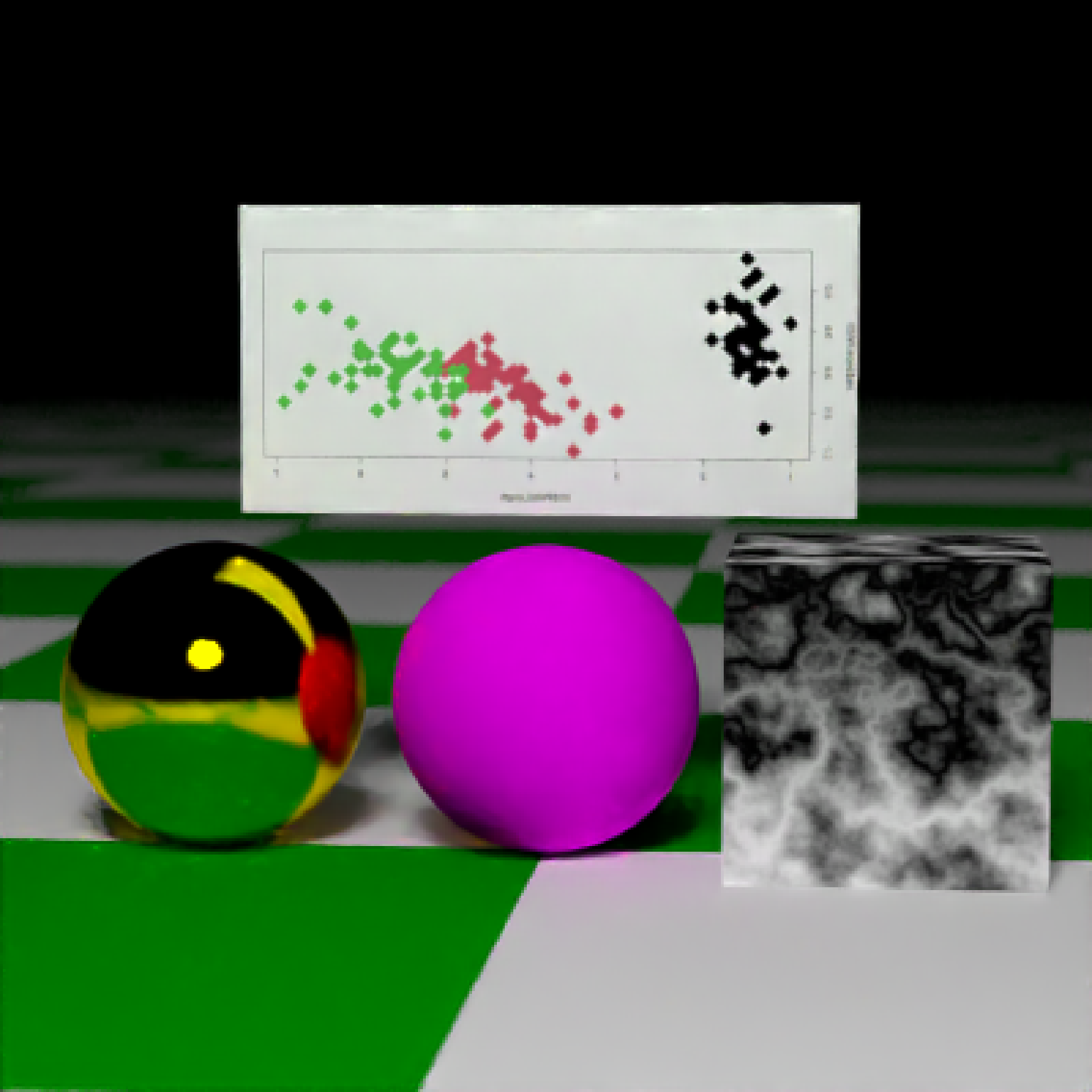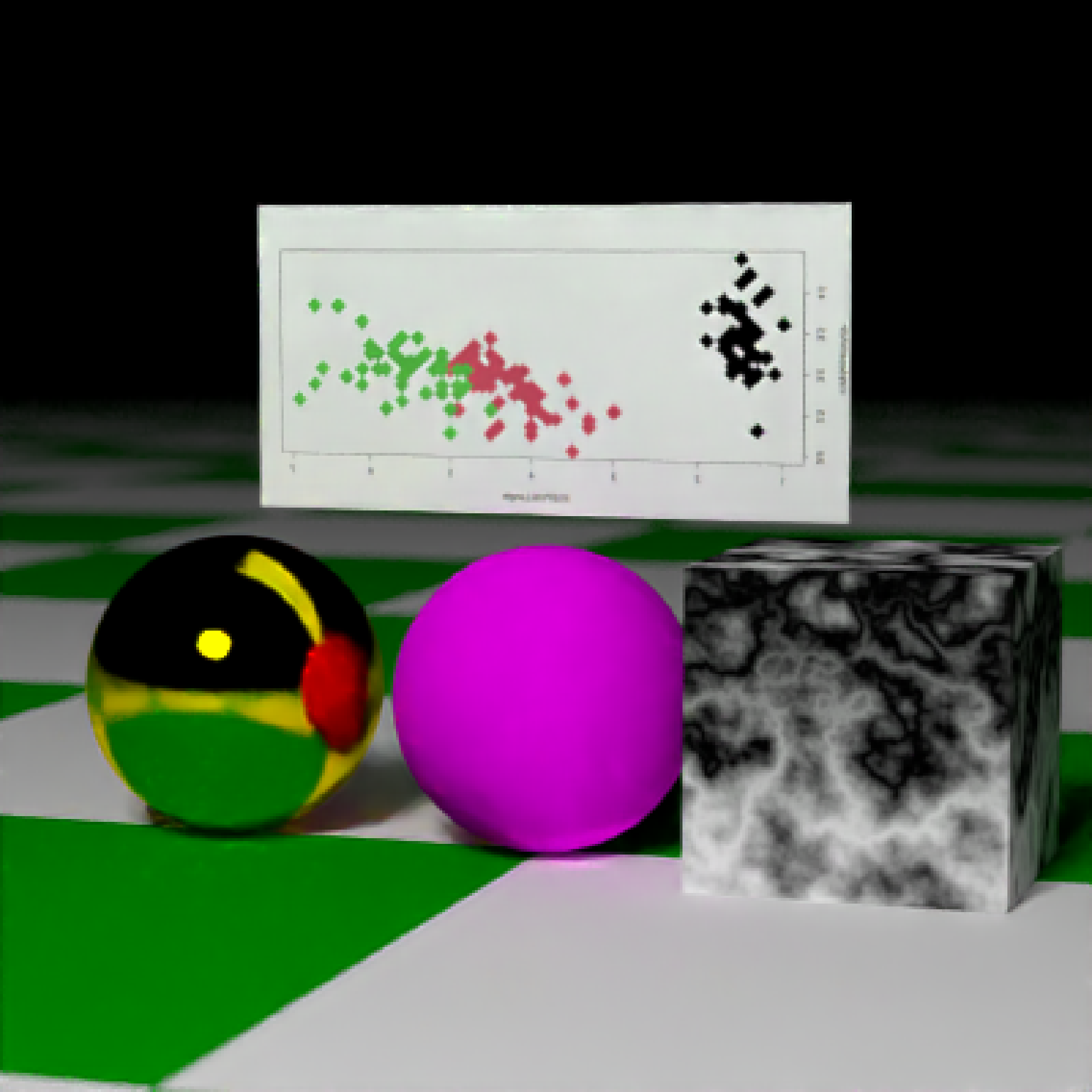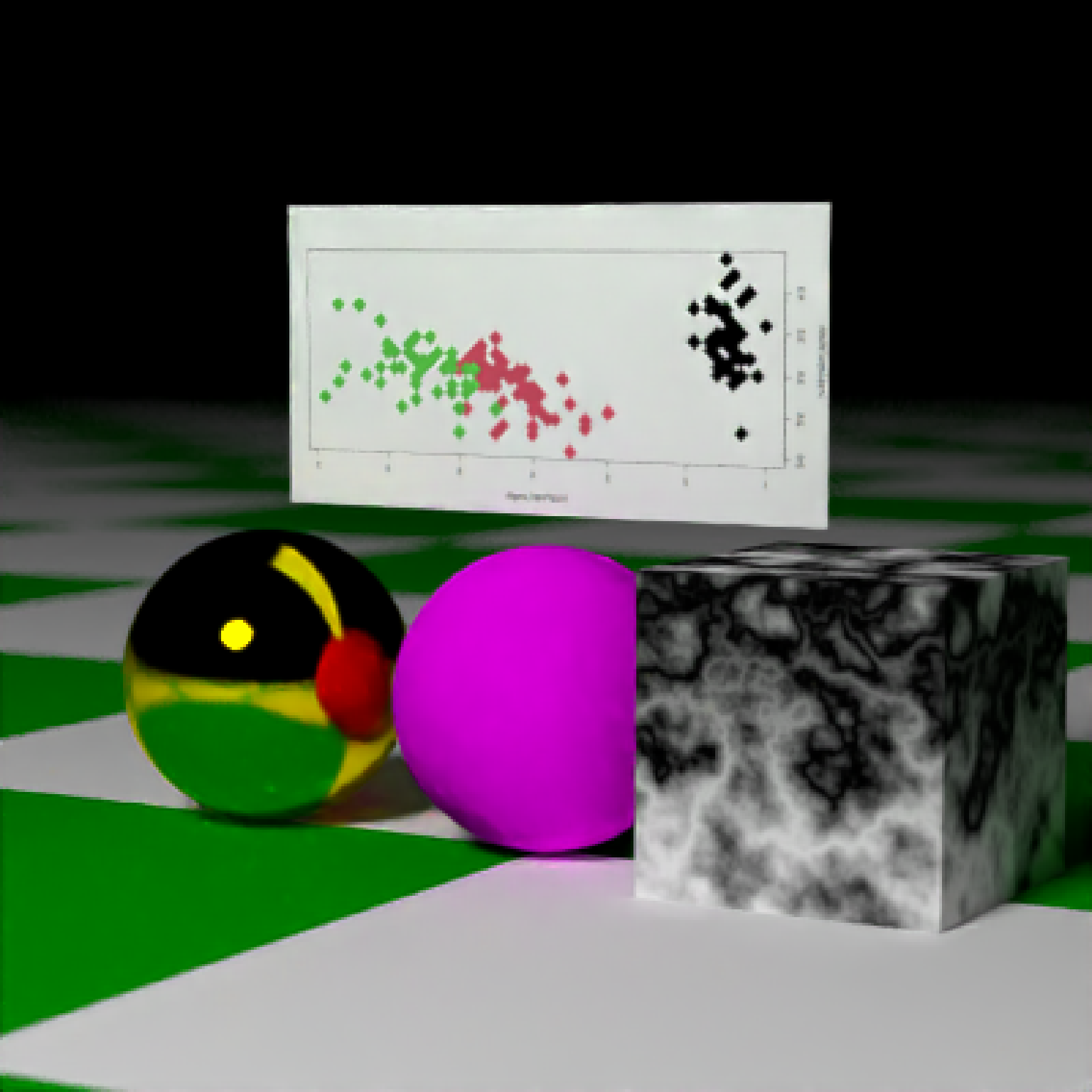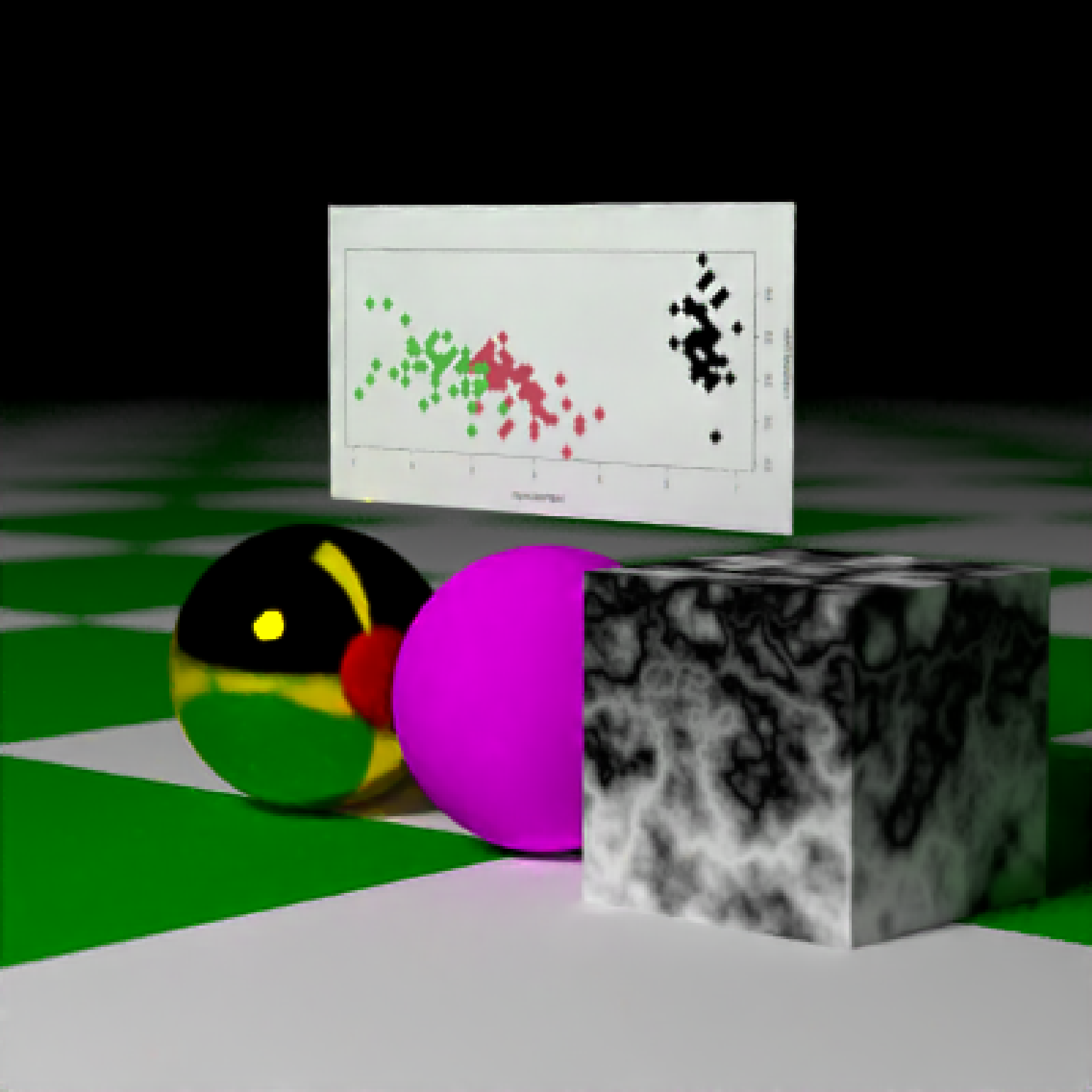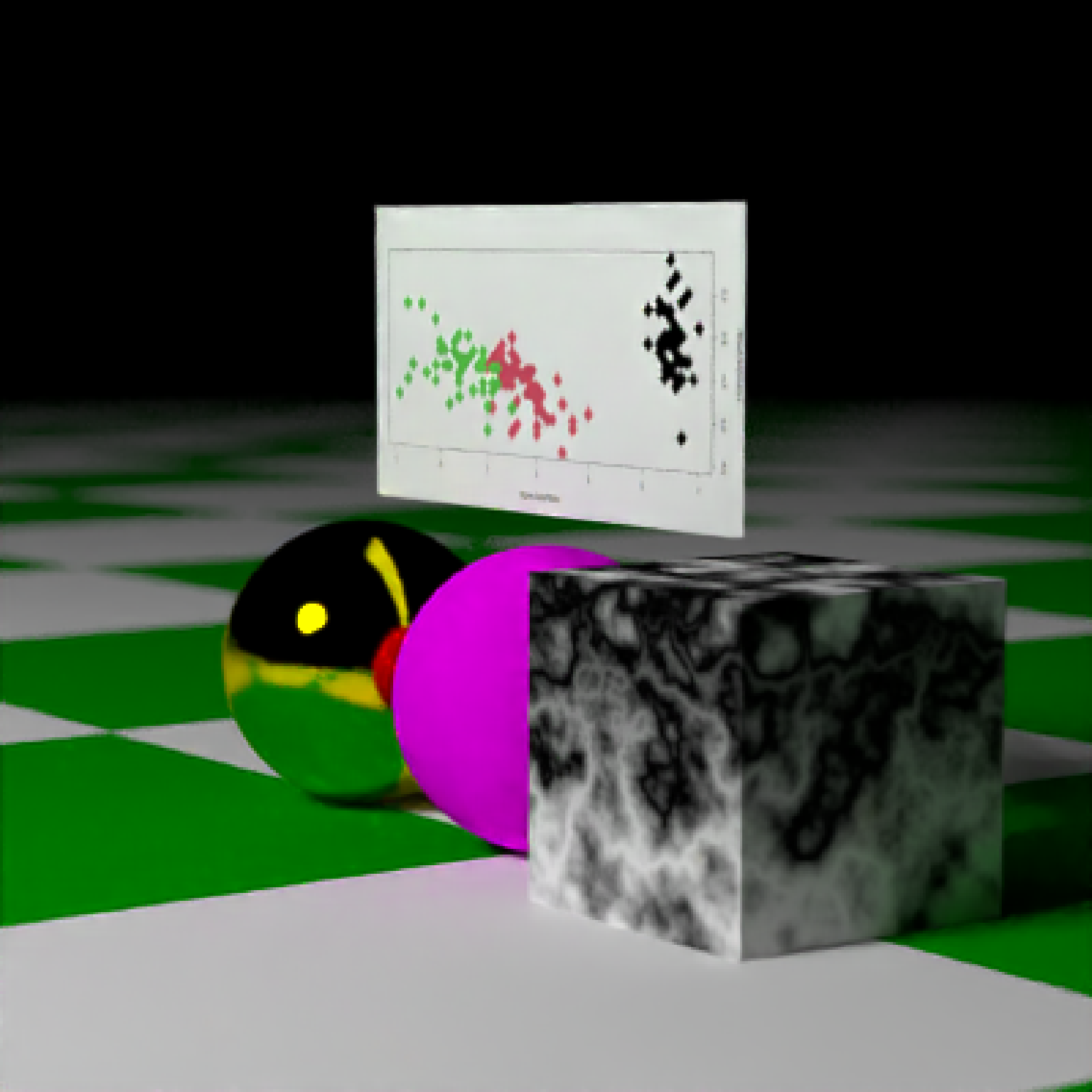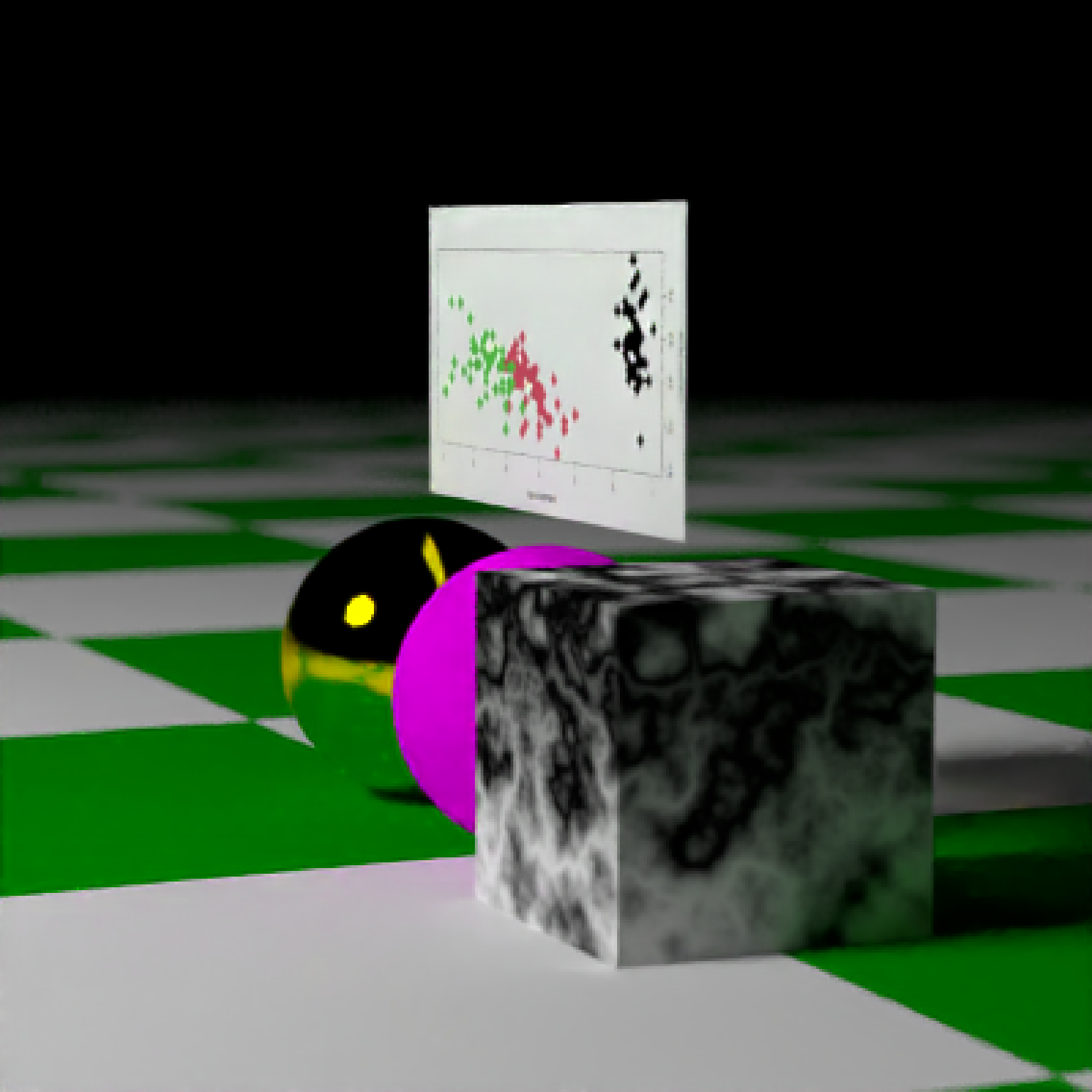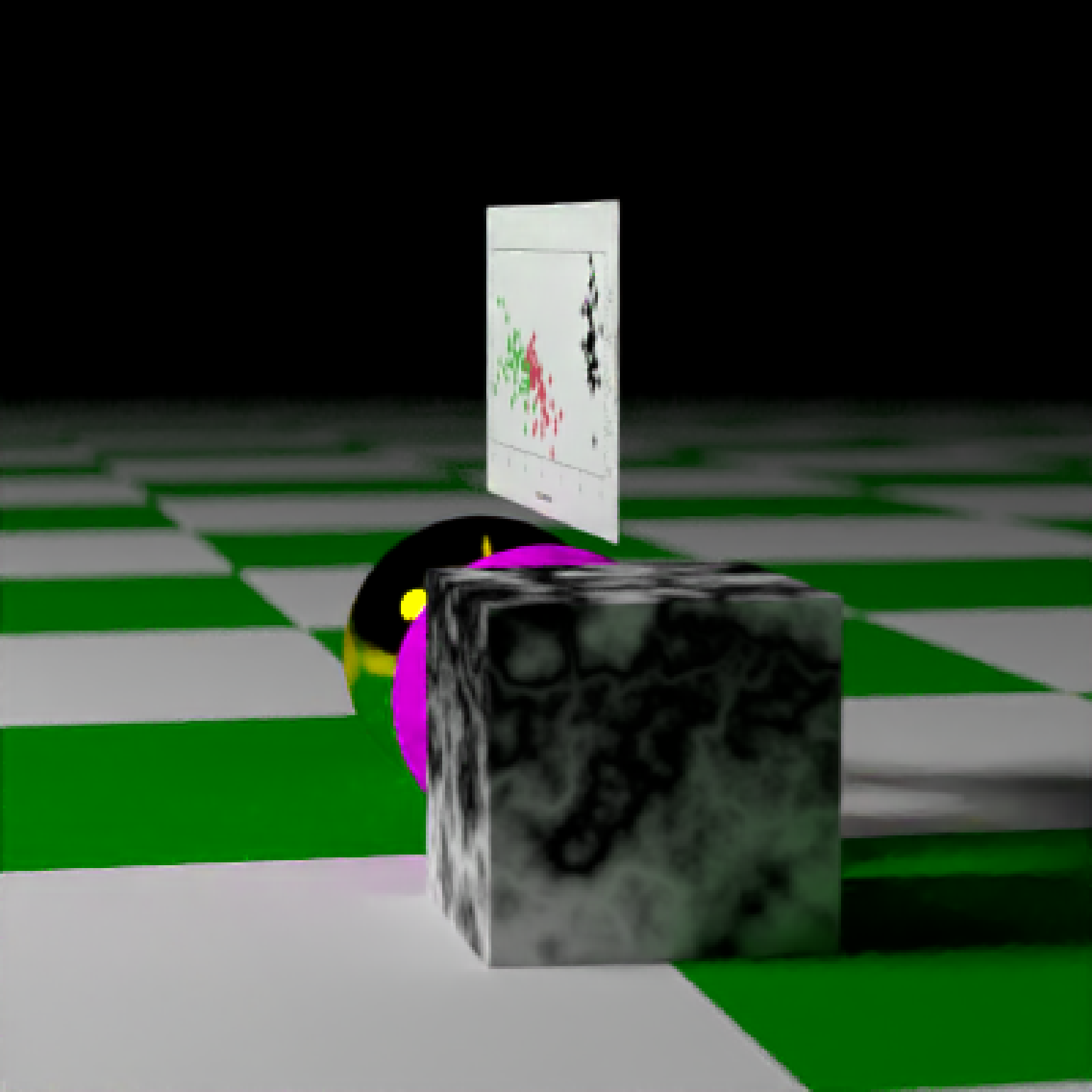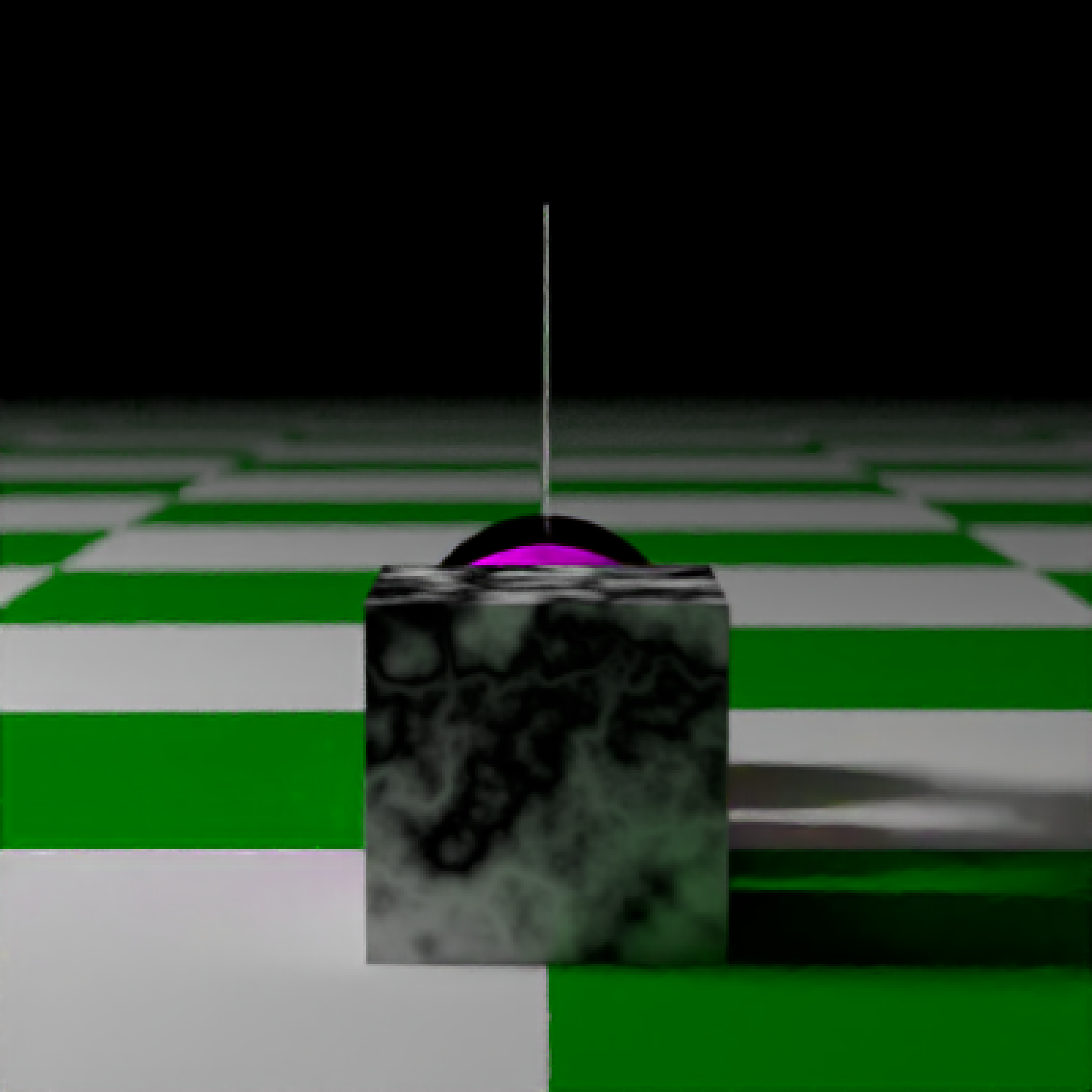Takes the scene description and renders an image, either to the device or to a filename. The user can also interactively fly around the 3D scene if they have X11 support on their system or are on Windows.

render_scene(
scene,
width = 400,
height = 400,
fov = 20,
samples = 100,
camera_description_file = NA,
preview = interactive(),
interactive = TRUE,
camera_scale = 1,
iso = 100,
film_size = 22,
min_variance = 5e-05,
sample_method = "sobol_blue",
max_depth = NA,
roulette_active_depth = 100,
ambient_light = NULL,
lookfrom = c(0, 1, 10),
lookat = c(0, 0, 0),
camera_up = c(0, 1, 0),
aperture = 0.1,
clamp_value = Inf,
filename = NULL,
backgroundhigh = "#80b4ff",
backgroundlow = "#ffffff",
shutteropen = 0,
shutterclose = 1,
focal_distance = NULL,
ortho_dimensions = c(1, 1),
tonemap = "gamma",
bloom = TRUE,
parallel = TRUE,
bvh_type = "sah",
environment_light = NULL,
rotate_env = 0,
intensity_env = 1,
transparent_background = FALSE,
debug_channel = "none",
return_raw_array = FALSE,
progress = interactive(),
verbose = FALSE,
new_page = TRUE
)

## Arguments

scene

Tibble of object locations and properties.

width

Default 400. Width of the render, in pixels.

height

Default 400. Height of the render, in pixels.

fov

Default 20. Field of view, in degrees. If this is 0, the camera will use an orthographic projection. The size of the plane used to create the orthographic projection is given in argument ortho_dimensions. From 0 to 180, this uses a perspective projections. If this value is 360, a 360 degree environment image will be rendered.

samples

Default 100. The maximum number of samples for each pixel. If this is a length-2 vector and the sample_method is stratified, this will control the number of strata in each dimension. The total number of samples in this case will be the product of the two numbers.

camera_description_file

Default NA. Filename of a camera description file for rendering with a realistic camera. Several camera files are built-in: "50mm","wide","fisheye", and "telephoto".

preview

Default TRUE. Whether to display a real-time progressive preview of the render. Press ESC to cancel the render.

interactive

Default interactive(). Whether the scene preview should be interactive. Camera movement orbits around the lookat point (unless the mode is switched to free flying), with the following control mapping: W = Forward, S = Backward, A = Left, D = Right, Q = Up, Z = Down, E = 2x Step Distance (max 128), C = 0.5x Step Distance, Up Key = Zoom In (decrease FOV), Down Key = Zoom Out (increase FOV), Left Key = Decrease Aperture, Right Key = Increase Aperture, 1 = Decrease Focal Distance, 2 = Increase Focal Distance, 3/4 = Rotate Environment Light, R = Reset Camera, TAB: Toggle Orbit Mode, Left Mouse Click: Change Look Direction, Right Mouse Click: Change Look At K: Save Keyframe (at the conclusion of the render, this will create the ray_keyframes data.frame in the global environment, which can be passed to generate_camera_motion() to tween between those saved positions. L: Reset Camera to Last Keyframe (if set) F: Toggle Fast Travel Mode

Initial step size is 1/20th of the distance from lookat to lookfrom.

Note: Clicking on the environment image will only redirect the view direction, not change the orbit point. Some options aren't available all cameras. When using a realistic camera, the aperture and field of view cannot be changed from their initial settings. Additionally, clicking to direct the camera at the background environment image while using a realistic camera will not always point to the exact position selected.

camera_scale

Default 1. Amount to scale the camera up or down in size. Use this rather than scaling a scene.

iso

Default 100. Camera exposure.

film_size

Default 22, in mm (scene units in m. Size of the film if using a realistic camera, otherwise ignored.

min_variance

Default 0.00005. Minimum acceptable variance for a block of pixels for the adaptive sampler. Smaller numbers give higher quality images, at the expense of longer rendering times. If this is set to zero, the adaptive sampler will be turned off and the renderer will use the maximum number of samples everywhere.

Default 8. Width of the minimum block size in the adaptive sampler.

sample_method

Default sobol. The type of sampling method used to generate random numbers. The other options are random (worst quality but fastest), stratified (only implemented for completion), sobol_blue (best option for sample counts below 256), and sobol (slowest but best quality, better than sobol_blue for sample counts greater than 256). If samples > 256 and sobol_blue is selected, the method will automatically switch to sample_method = "sobol".

max_depth

Default NA, automatically sets to 50. Maximum number of bounces a ray can make in a scene. Alternatively, if a debugging option is chosen, this sets the bounce to query the debugging parameter (only for some options).

roulette_active_depth

Default 100. Number of ray bounces until a ray can stop bouncing via Russian roulette.

ambient_light

Default FALSE, unless there are no emitting objects in the scene. If TRUE, the background will be a gradient varying from backgroundhigh directly up (+y) to backgroundlow directly down (-y).

lookfrom

Default c(0,1,10). Location of the camera.

lookat

Default c(0,0,0). Location where the camera is pointed.

camera_up

Default c(0,1,0). Vector indicating the "up" position of the camera.

aperture

Default 0.1. Aperture of the camera. Smaller numbers will increase depth of field, causing less blurring in areas not in focus.

clamp_value

Default Inf. If a bright light or a reflective material is in the scene, occasionally there will be bright spots that will not go away even with a large number of samples. These can be removed (at the cost of slightly darkening the image) by setting this to a small number greater than 1.

filename

Default NULL. If present, the renderer will write to the filename instead of the current device.

backgroundhigh

Default #80b4ff. The "high" color in the background gradient. Can be either a hexadecimal code, or a numeric rgb vector listing three intensities between 0 and 1.

backgroundlow

Default #ffffff. The "low" color in the background gradient. Can be either a hexadecimal code, or a numeric rgb vector listing three intensities between 0 and 1.

shutteropen

Default 0. Time at which the shutter is open. Only affects moving objects.

shutterclose

Default 1. Time at which the shutter is open. Only affects moving objects.

focal_distance

Default NULL, automatically set to the lookfrom-lookat distance unless otherwise specified.

ortho_dimensions

Default c(1,1). Width and height of the orthographic camera. Will only be used if fov = 0.

tonemap

Default gamma. Choose the tone mapping function, Default gamma solely adjusts for gamma and clamps values greater than 1 to 1. reinhold scales values by their individual color channels color/(1+color) and then performs the gamma adjustment. uncharted uses the mapping developed for Uncharted 2 by John Hable. hbd uses an optimized formula by Jim Hejl and Richard Burgess-Dawson. If raw, the raw array of HDR values will be returned, rather than an image or a plot.

bloom

Default TRUE. Set to FALSE to get the raw, pathtraced image. Otherwise, this performs a convolution of the HDR image of the scene with a sharp, long-tailed exponential kernel, which does not visibly affect dimly pixels, but does result in emitters light slightly bleeding into adjacent pixels. This provides an antialiasing effect for lights, even when tonemapping the image. Pass in a matrix to specify the convolution kernel manually, or a positive number to control the intensity of the bloom (higher number = more bloom).

parallel

Default TRUE. If FALSE, it will use all available cores to render the image (or the number specified in options("cores") or options("Ncpus") if that option is not NULL).

bvh_type

Default "sah", "surface area heuristic". Method of building the bounding volume hierarchy structure used when rendering. Other option is "equal", which splits tree into groups of equal size.

environment_light

Default NULL. An image to be used for the background for rays that escape the scene. Supports both HDR (.hdr) and low-dynamic range (.png, .jpg) images.

rotate_env

Default 0. The number of degrees to rotate the environment map around the scene.

intensity_env

Default 1. The amount to increase the intensity of the environment lighting. Useful if using a LDR (JPEG or PNG) image as an environment map.

transparent_background

Default FALSE. If TRUE, any initial camera rays that escape the scene will be marked as transparent in the final image. If for a pixel some rays escape and others hit a surface, those pixels will be partially transparent.

debug_channel

Default none. If depth, function will return a depth map of rays into the scene instead of an image. If normals, function will return an image of scene normals, mapped from 0 to 1. If uv, function will return an image of the uv coords. If variance, function will return an image showing the number of samples needed to take for each block to converge. If dpdu or dpdv, function will return an image showing the differential u and u coordinates. If color, function will return the raw albedo values (with white for metal and dielectric materials).

return_raw_array

Default FALSE. If TRUE, function will return raw array with RGB intensity information.

progress

Default TRUE if interactive session, FALSE otherwise.

verbose

Default FALSE. Prints information and timing information about scene construction and raytracing progress.

new_page

Default TRUE. Whether to call grid::grid.newpage() when plotting the image (if no filename specified). Set to FALSE for faster plotting (does not affect render time).

## Value

A pathtraced image to the current device, or an image saved to a file. Invisibly returns the array (containing either debug data or the RGB).

## Examples

# Generate a large checkered sphere as the ground
if (run_documentation()) {
scene = generate_ground(depth = -0.5,
material = diffuse(color = "white", checkercolor = "darkgreen"))
render_scene(scene, parallel = TRUE, samples = 128, sample_method = "sobol")
}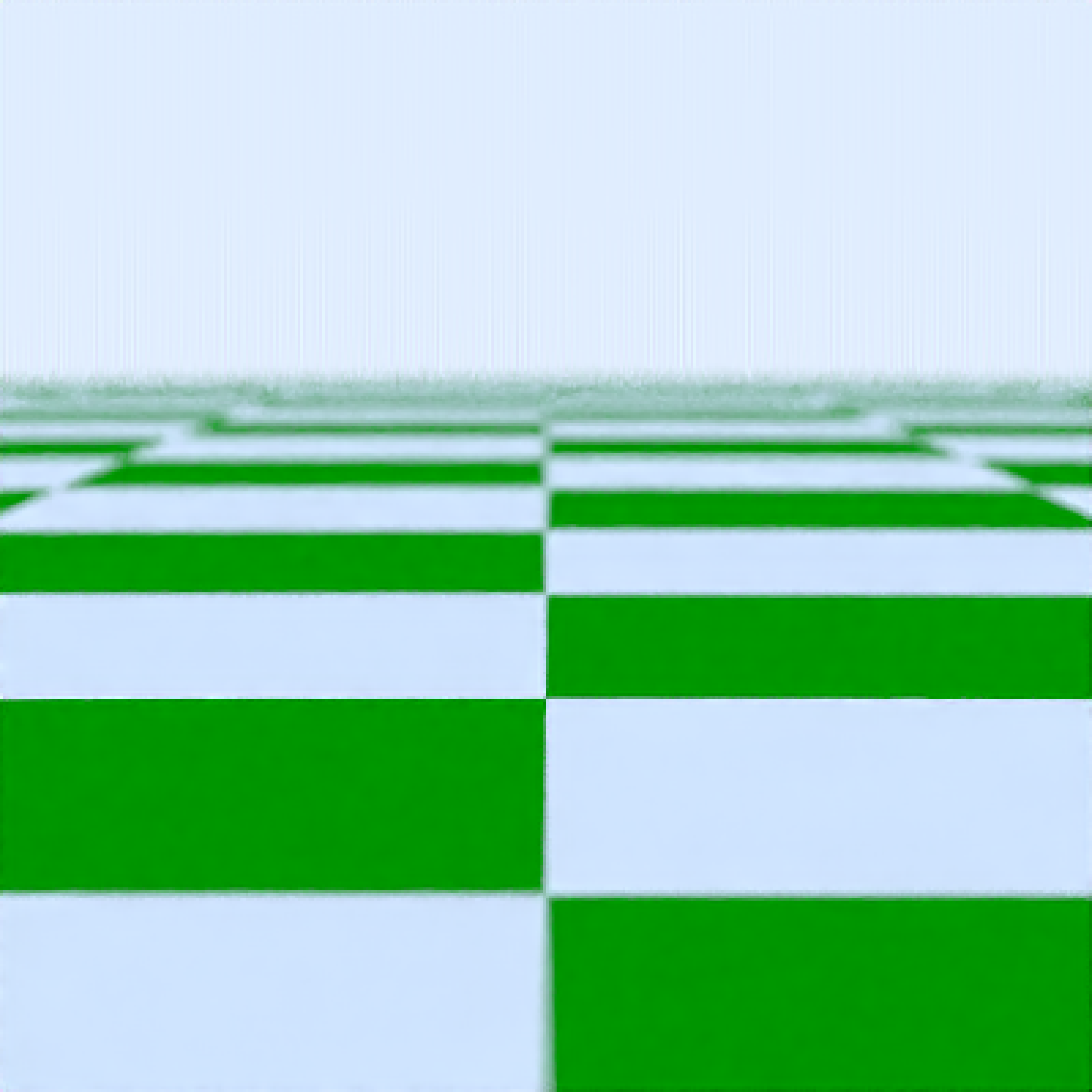if (run_documentation()) {
# Add a sphere to the center
scene = scene %>%
add_object(sphere(x = 0, y = 0, z = 0, radius = 0.5, material = diffuse(color = c(1, 0, 1))))
render_scene(scene, fov = 20, parallel = TRUE, samples = 128)
}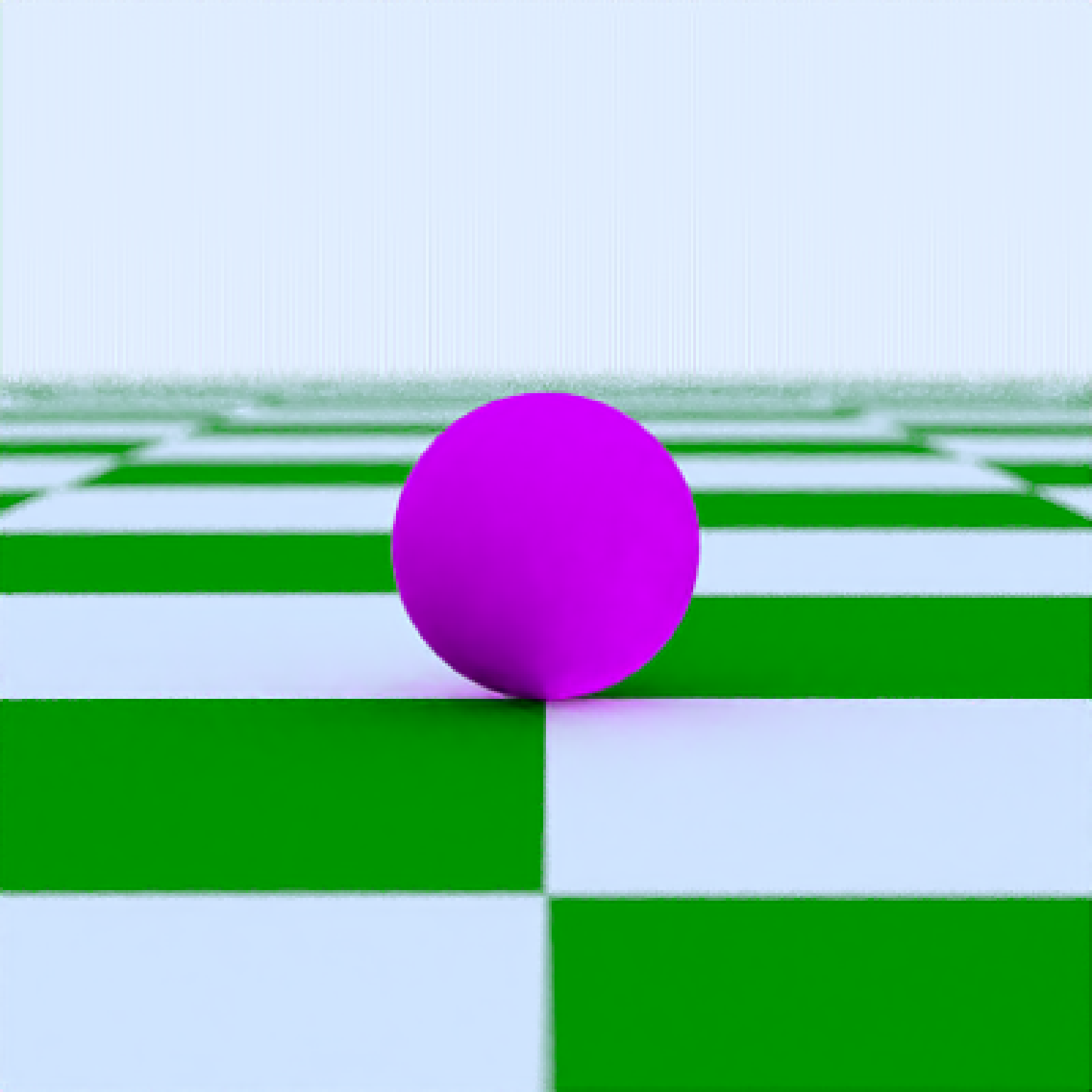if (run_documentation()) {
scene = scene %>%
add_object(cube(x = 1.1, y = 0, z = 0, material = diffuse(noise = 3)))
render_scene(scene, fov = 20, parallel = TRUE, samples = 128)
}if (run_documentation()) {
# Add a metallic gold sphere, using stratified sampling for a higher quality render
# We also add a light, which turns off the default ambient lighting
scene = scene %>%
add_object(sphere(x = -1.1, y = 0, z = 0, radius = 0.5,
material = metal(color = "gold", fuzz = 0.1))) %>%
render_scene(scene, fov = 20, parallel = TRUE, samples = 128)
}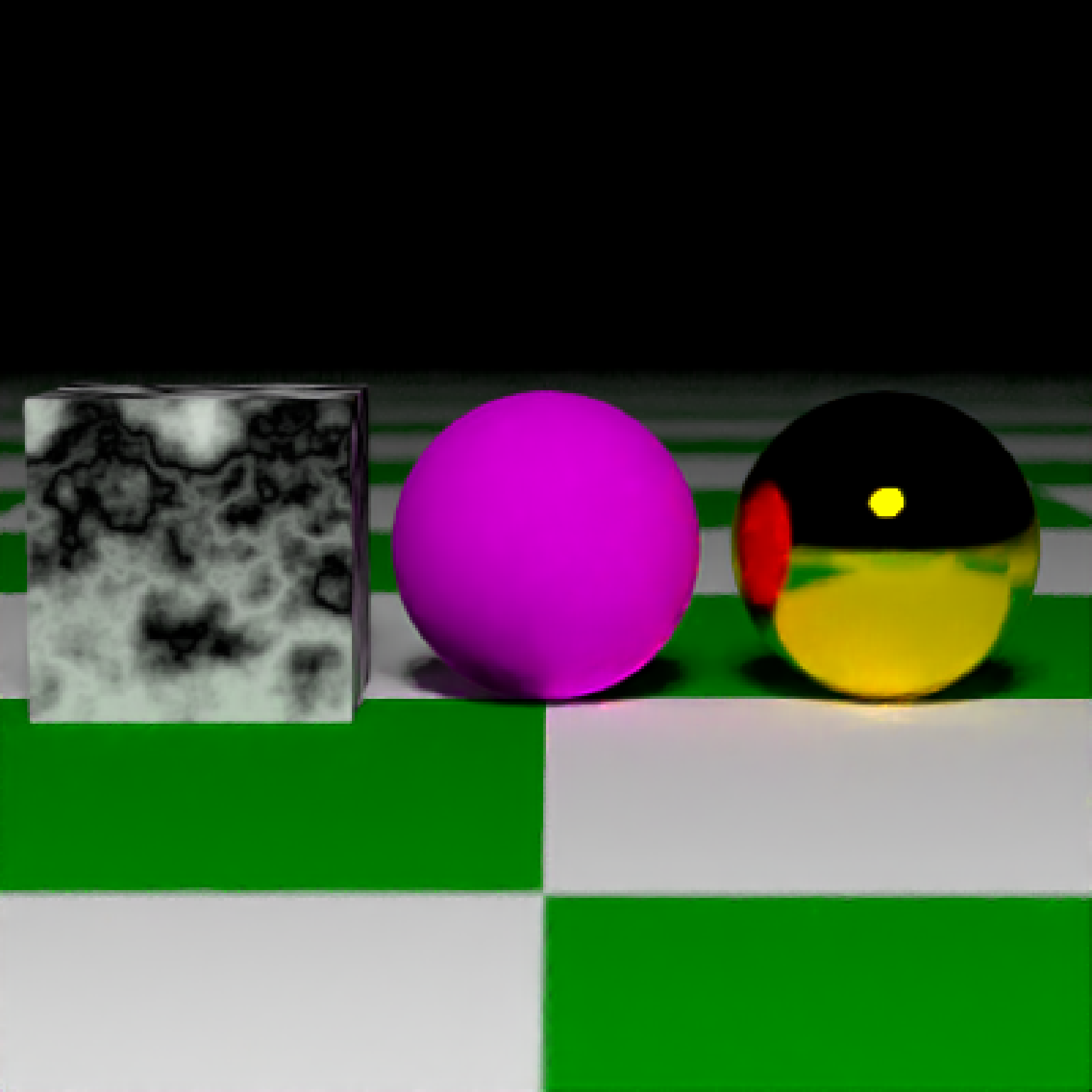if (run_documentation()) {
# Lower the number of samples to render more quickly (here, we also use only one core).
render_scene(scene, samples = 4, parallel = FALSE)
}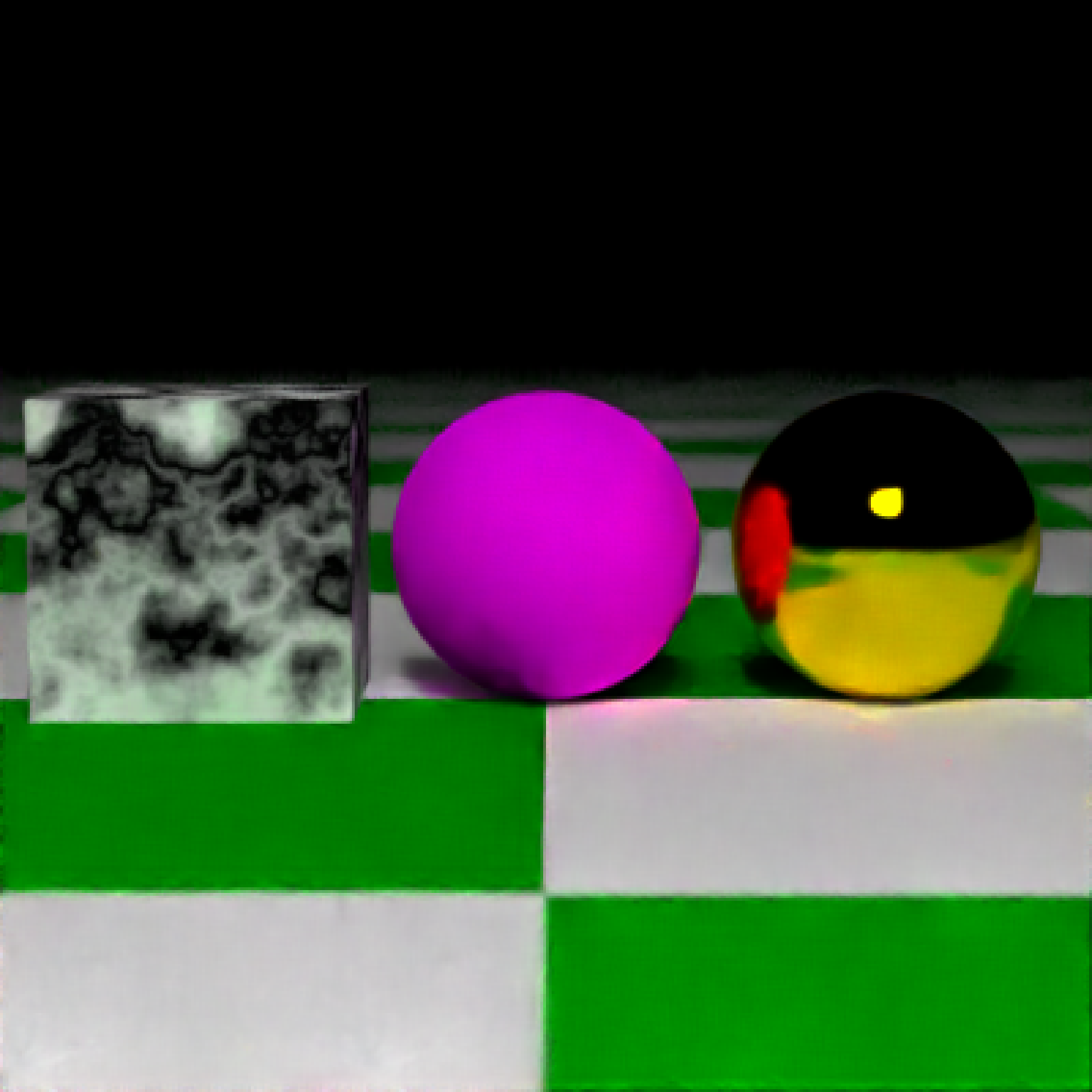if (run_documentation()) {
# Add a floating R plot using the iris dataset as a png onto a floating 2D rectangle
tempfileplot = tempfile()
png(filename = tempfileplot, height = 400, width = 800)
plot(iris$Petal.Length, iris$Sepal.Width, col = iris\$Species, pch = 18, cex = 4)
dev.off()
image_array = aperm(png::readPNG(tempfileplot), c(2, 1, 3))
scene = scene %>%
add_object(xy_rect(x = 0, y = 1.1, z = 0, xwidth = 2, angle = c(0, 0, 0),
material = diffuse(image_texture = image_array)))
render_scene(scene, fov = 20, parallel = TRUE, samples = 128)
}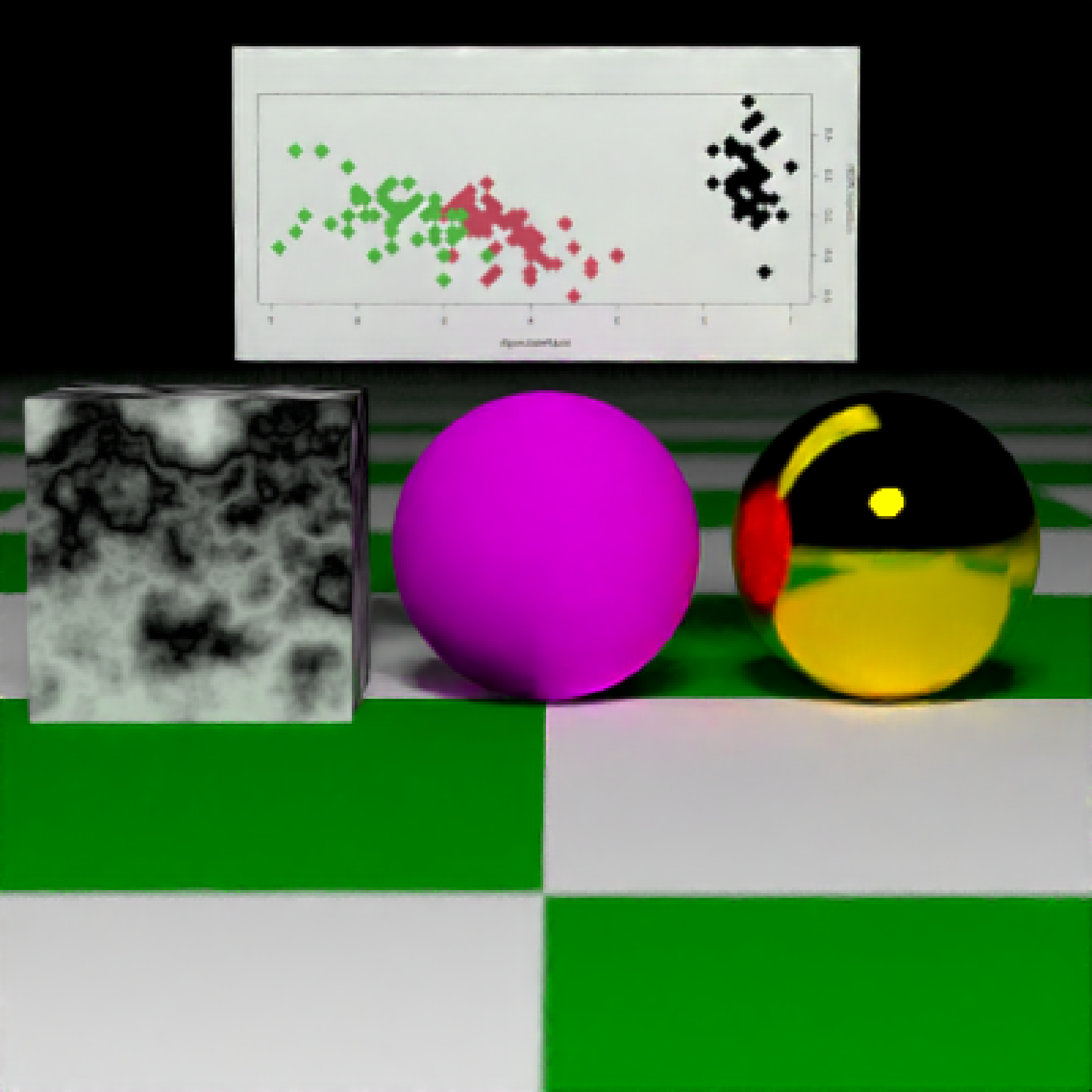if (run_documentation()) {
# Move the camera
render_scene(scene, lookfrom = c(7, 1.5, 10), lookat = c(0, 0.5, 0), fov = 15, parallel = TRUE)
}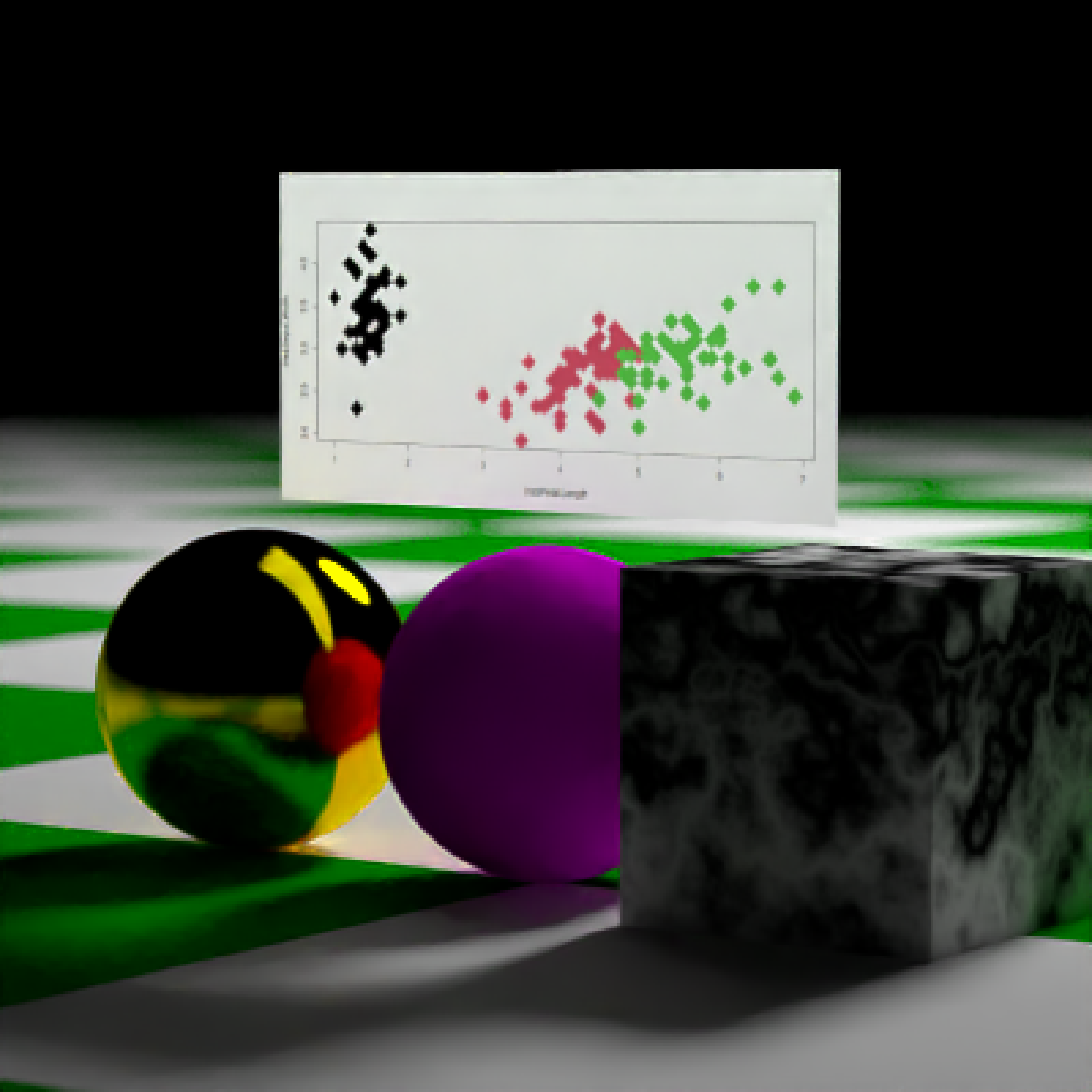if (run_documentation()) {
# Change the background gradient to a firey sky
render_scene(scene, lookfrom = c(7, 1.5, 10), lookat = c(0, 0.5, 0), fov = 15,
backgroundhigh = "orange", backgroundlow = "red", parallel = TRUE,
ambient = TRUE,
samples = 128)
}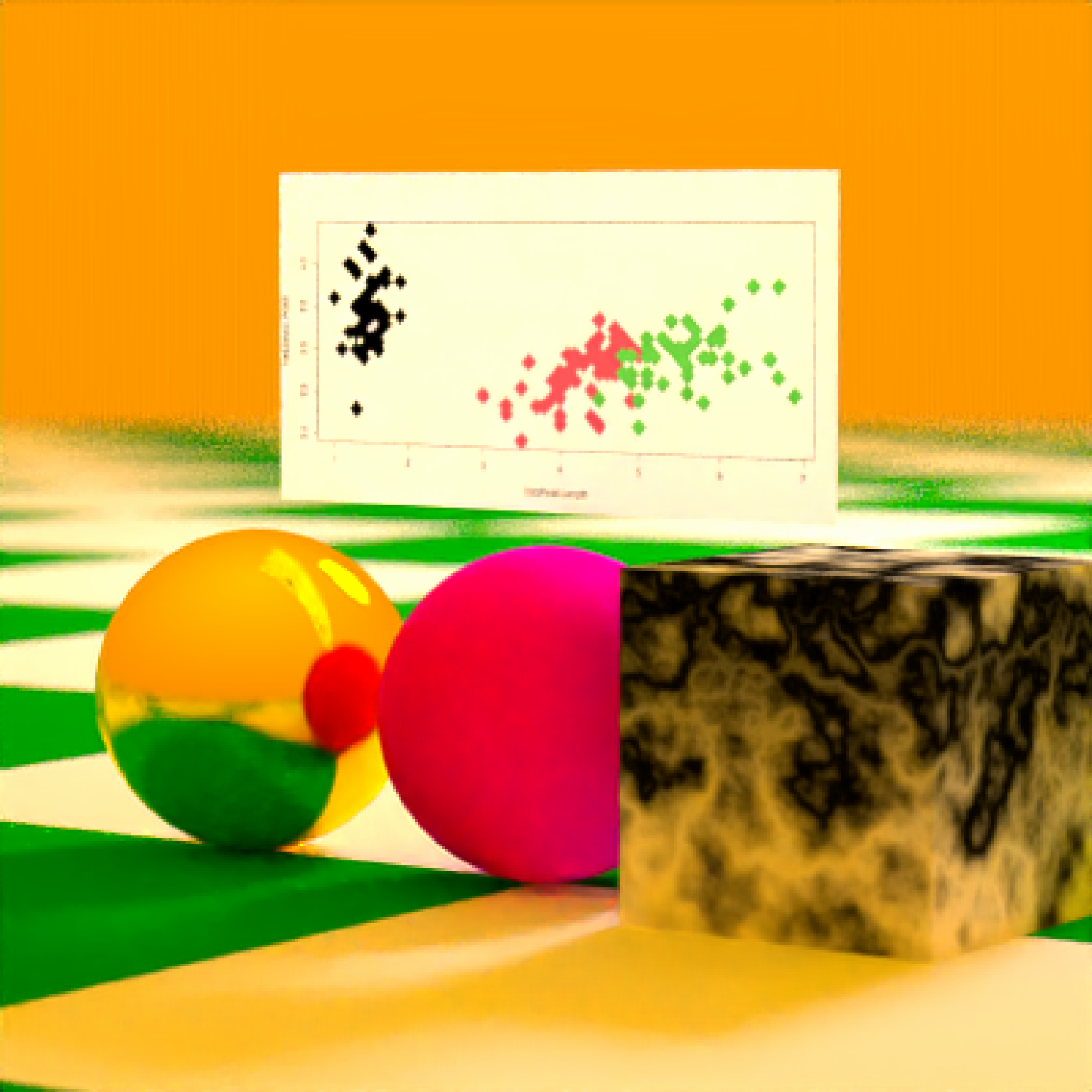if (run_documentation()) {
# Increase the aperture to blur objects that are further from the focal plane.
render_scene(scene, lookfrom = c(7, 1.5, 10), lookat = c(0, 0.5, 0), fov = 15,
aperture = 1, parallel = TRUE, samples = 128)
}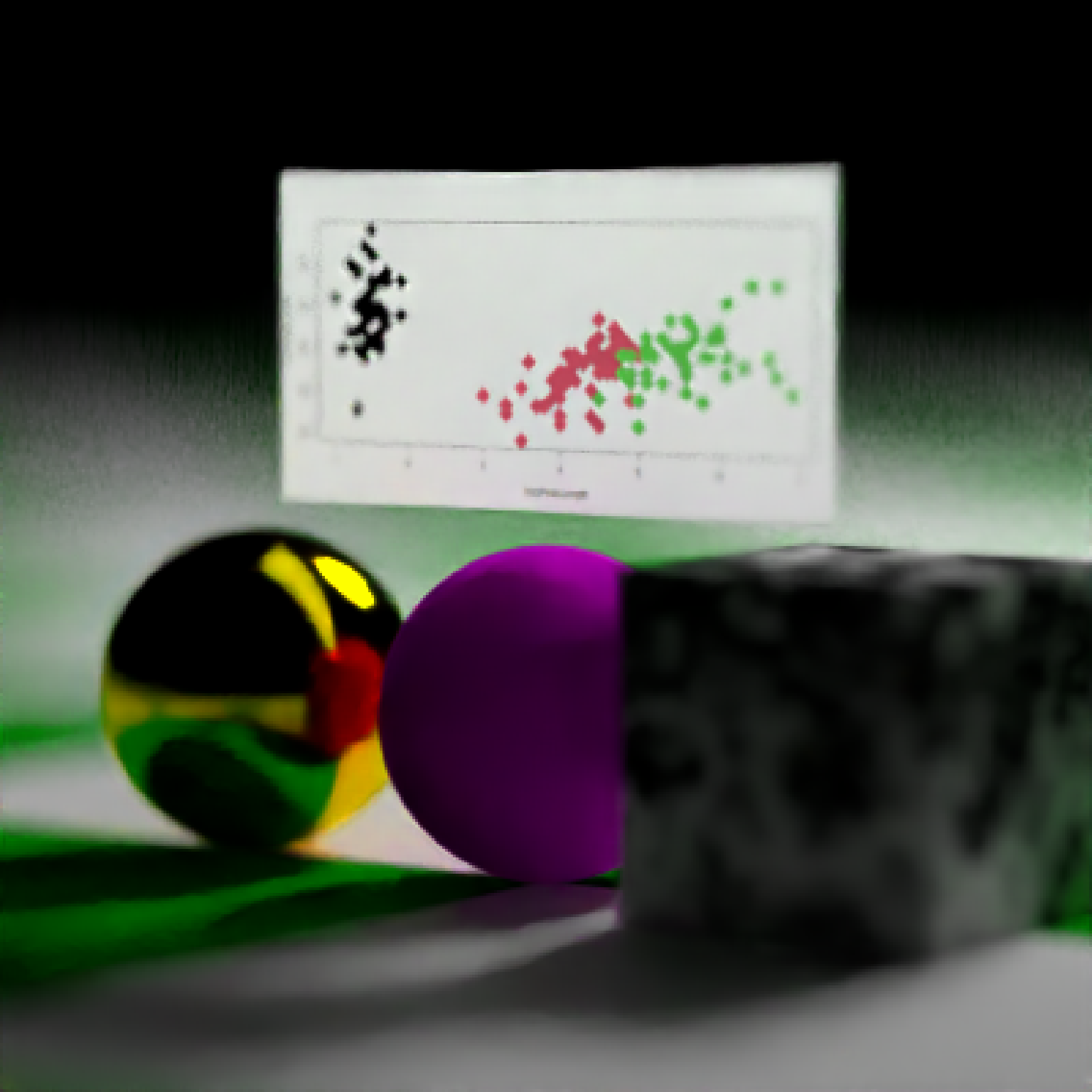if (run_documentation()) {
# We can also capture a 360 environment image by setting fov = 360 (can be used for VR)
generate_cornell() %>%
add_object(ellipsoid(x = 555 / 2, y = 100, z = 555 / 2, a = 50, b = 100, c = 50,
material = metal(color = "lightblue"))) %>%
add_object(cube(x = 100, y = 130 / 2, z = 200, xwidth = 130, ywidth = 130, zwidth = 130,
material = diffuse(checkercolor = "purple",
checkerperiod = 30), angle = c(0, 10, 0))) %>%
add_object(pig(x = 100, y = 190, z = 200, scale = 40, angle = c(0, 30, 0))) %>%
add_object(sphere(x = 420, y = 555 / 8, z = 100, radius = 555 / 8,
material = dielectric(color = "orange"))) %>%
add_object(xz_rect(x = 555 / 2, z = 555 / 2, y = 1, xwidth = 555, zwidth = 555,
material = glossy(checkercolor = "white",
checkerperiod = 10, color = "dodgerblue"))) %>%
render_scene(lookfrom = c(278, 278, 30), lookat = c(278, 278, 500), clamp_value = 10,
fov = 360,  samples = 128, width = 800, height = 800)
}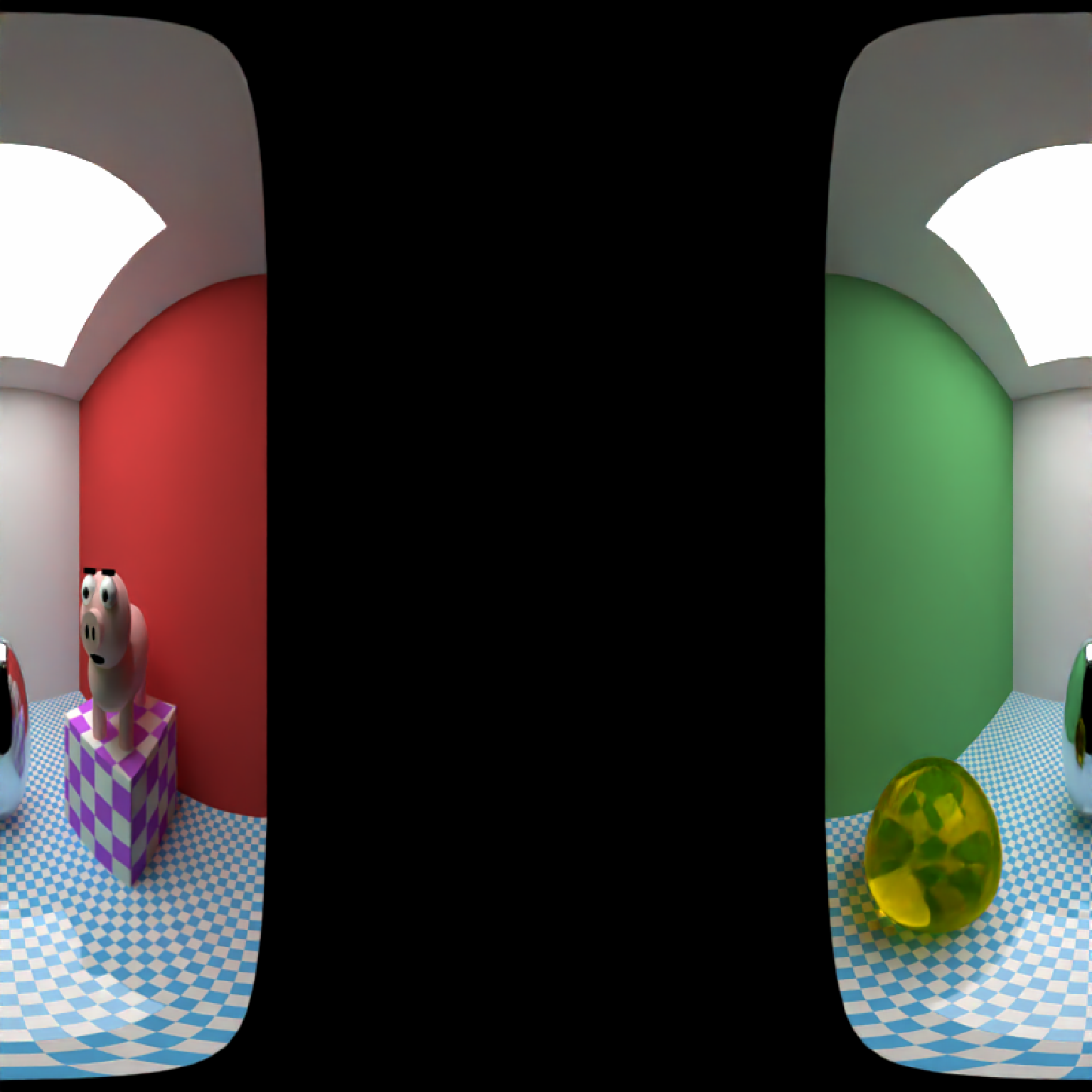if (run_documentation()) {
# Spin the camera around the scene, decreasing the number of samples to render faster. To make
# an animation, specify the a filename in render_scene for each frame and use the av package
# or ffmpeg to combine them all into a movie.
t = 1:30
xpos = 10 * sin(t * 12 * pi / 180 + pi / 2)
zpos = 10 * cos(t * 12 * pi / 180 + pi / 2)
# Save old par() settings
on.exit(par(old.par))
par(mfrow = c(5, 6))
for (i in 1:30) {
render_scene(scene, samples = 16,
lookfrom = c(xpos[i], 1.5, zpos[i]), lookat = c(0, 0.5, 0), parallel = TRUE)
}
}